# A single neuron perceptron with sigmoid activation function – III – two ways of applying Normalizer

In this article series on a perceptron with only one computing neuron we saw that saturation effects of the sigmoid activation function can hamper gradient descent if input data on some features become too big and/or the initial weight distribution is not adapted to the number of input features. See:

A single neuron perceptron with sigmoid activation function – I – failure of gradient descent due to saturation

We can remedy the first point by applying a normalization transformation to the input data before starting gradient descent. I showed the positive result of such a transformation for our perceptron with a rather specific set of input data in the last article:

A single neuron perceptron with sigmoid activation function – II – normalization to overcome saturation

At that time we used the "StandardScaler" provided by Scikit-Learn. In this article we shall instead use an instance of the "Normalizer" class for scaling. With "Normalizer" you have to be a bit careful how you use its interface. We shall apply "Normalizer in two different ways. Besides having some fun with the outcome, we will also learn that the shape of the clusters in which the input samples may be arranged in feature space should be taken into account before normalizing ahead of classification tasks. Which may be difficult in multiple dimensions ... but it brings us to the general idea of identifying a method of cluster identification ahead of classification training with gradient descent.

# How does the "Normalizer" work?

Let us offer a "Normalizer"-instance an input array "ay_in" with 2 rows and 4 columns for each row. The shape of "ay_in" is (2,4). The first row "s1" shall have elements like s1=[4, 1, 2, 2]. Then Normalizer will then calculate a L2-norm value for the column data of our specific row as

L2([4, 1, 2, 2]) = sqrt(4**2 + 1**2 + 2**2 + 2**2) = 5
=> s1_trafo = [4/L2, 1/L2, 2/L2, 2/L2] = [0.8, 0.2, 0.4, 0.4].

I.e., all columns in one row are multiplied by one common factor determined as the L2-norm of the column data of the sample. Note again: Each row is treated separately. So, an array as

```[
[1, 3, 9, 3],
[5, 7, 5, 1]
]
```

will be transformed to

```  [0.1,, 0.3, 0.9, 0.3],
[0.5, 0.7, 0.5, 0.1]
]
```

How can we make use of this for our perceptron samples?

# Standard scaling per feature with Normalizer

A first idea is that we could scale the data of all samples for our perceptron separately per feature; i.e. we collect the data-values of all M samples for "feature 1" in an array and offer it as the first row of an array to Normalizer, plus a row with all the data values for "feature 2", .... and so on.

If we had M samples and N features we would present an array with shape (N, M) to "Normalizer". In our simple perceptron experiment this is equivalent to scaling data of an array where the two rows are defined by our K1 and K2-input arrays => ay_K = [ li_K1, li_K2 ].

What would the outcome of such a scaling be?

A constant factor per feature determined by the L2-norm of all samples' values for the chosen feature brings all values safely down into an interval of [-1, 1]. But this also means that the maximum value of all samples for a specific feature determines the scale.

Then so called "outliers", i.e. samples whose values are far away from the average values of the samples, would have a major impact. So "Normalizer"-Scaling is especially helpful, if the values per feature are limited by principle reasons. Note that this is e.g. the case with RGB-color or gray-scale values! Note also that the possible impact of outliers is also relevant for other normalizers as the "MinMaxNormalizer" of SciKit-Learn.

Although the scaling factors will be different per feature I would like to point out another aspect of scaling by a constant factor per feature over all samples: Such a transformation keeps up at least some structural similarity of the sample distribution in the feature space.

# Scaling features per sample with Normalizer (?)

A different way of applying "Normalizer" would be to use the transformed array "ay_K.T" as input: For M samples and N features we would then present an array with shape (M, N) to a Normalizer instance. Its algorithm would then scale across the features of each sample. If we interpret a specific sample as a vector in the feature space then the L2-norm corresponds naturally to the length of this vector. Meaning: Normalizer would scale each sample by its vector length.

# Two questions before experimenting

The two possible application methods for Normalizer lead directly to two questions for our simple test setup in a 2-dim feature space:

• How will lines of equal cost values (i.e. cost or loss contours) for our sigmoid-based loss function look like in the {K1, K2}-space after scaling a bunch of N (K1, K2)-datapoints with Normalizer per feature? I.e., if and when should we present an array of feature values with shape (N,M)?
• What would happen instead if we scaled each input sample individually across its features? I.e., what happens in a situation with M samples and N features and we feed "Normalizer" with an array (of the same feature values) which has a shape (N,M) instead of (M,N)?

I guess a "natural talent" on numbers as Mr Trump could give the answers without hesitation 🙂 . As we certainly are below the standards of the "genius" Mr Trump (his own words on multiple occasions) we shall pick the answers from plots below before we even try a deeper reasoning.

# Application of "Normalizer" separately to the feature data of all batch samples

As you remember from the first article of this series our input batch contained samples (K1, K2) with values for K1 and K2 given by two 1-dim arrays :

```li_K1 = [200.0,   1.0, 160.0,  11.0, 220.0,  11.0, 120.0,  22.0, 195.0,  15.0, 130.0,   5.0, 185.0,  16.0]
li_K2 = [ 14.0, 107.0,  10.0, 193.0,  32.0, 178.0,   2.0, 210.0,  12.0, 134.0,  15.0, 167.0,  10.0, 229.0]
```

The standard scaling application of Normalizer can be coded explicitly as (see the code given in the last article):

```    rg_idx = range(num_samples)
if scale_method == 0:
shape_input = (2, num_samples)
ay_K = np.zeros(shape_input)
for idx in rg_idx:
ay_K[idx] = li_K1[idx]
ay_K[idx] = li_K2[idx]
scaler = Normalizer()
ay_K = scaler.fit_transform(ay_K)
for idx in rg_idx:
ay_K1[idx] = ay_K[idx]
ay_K2[idx] = ay_K[idx]
scaling_fact_K1 = ay_K1 / li_K1
scaling_fact_K2 = ay_K2 / li_K2
print(ay_K1)
print("\n")
print(ay_K2)

```

However, a much faster form, which avoids the explicit Python loop, is given by:

```ay_K= np.vstack( (li_K1, li_K2) )
ay_K = scaler.fit_transform(ay_K)
ay_K1, ay_K2 = ay_K
scaling_fact_K1 = ay_K1 / li_K1
scaling_fact_K2 = ay_K2 / li_K2
```

Here OpenBlas helps 🙂 .

In contrast to other scalers we need to save and keep the factors by which we transform the various feature data by ourselves somewhere. (This is clear as "Normalizer" calculates a different factor for each feature.) So, we change our Jupyter cell code for scaling to:

```# ********
# Scaling
# ********

b_scale = True
scale_method = 0
# 0: Normalizer (standard), 1: StandardScaler, 2. By factor, 3: Normalizer per pair
# 4: Min_Max, 5: Identity (no transformation) - just there for convenience

shape_ay = (num_samples,)
ay_K1 = np.zeros(shape_ay)
ay_K2 = np.zeros(shape_ay)

# apply scaling
if b_scale:
# shape_input = (num_samples,2)
rg_idx = range(num_samples)
if scale_method == 0:
ay_K = np.vstack( (li_K1, li_K2) )
print("ay_k.shape = ", ay_K.shape)
scaler = Normalizer()
ay_K = scaler.fit_transform(ay_K)
ay_K1, ay_K2 = ay_K
scaling_fact_K1 = ay_K1 / li_K1
scaling_fact_K2 = ay_K2 / li_K2
print("\nay_K1 = \n", ay_K1)
print("\nay_K2 = \n", ay_K2)
print("\nscaling_fact_K1: ", scaling_fact_K1, ", scaling_fact_K2: ", scaling_fact_K2)

elif scale_method == 1:
ay_K = np.column_stack((li_K1, li_K2))
scaler = StandardScaler()
ay_K = scaler.fit_transform(ay_K)
ay_K1, ay_K2 = ay_K.T

elif scale_method == 2:
dmax = max(li_K1.max() - li_K1.min(), li_K2.max() - li_K2.min())
ay_K1 = 1.0/dmax * li_K1
ay_K2 = 1.0/dmax * li_K2
scaling_fact_K1 = ay_K1 / li_K1
scaling_fact_K2 = ay_K2 / li_K2

elif scale_method == 3:
ay_K = np.column_stack((li_K1, li_K2))
scaler = Normalizer()
ay_K = scaler.fit_transform(ay_K)
ay_K1, ay_K2 = ay_K.T

elif scale_method == 4:
ay_K = np.column_stack((li_K1, li_K2))
scaler = MinMaxScaler()
ay_K = scaler.fit_transform(ay_K)
ay_K1, ay_K2 = ay_K.T

elif scale_method == 5:
ay_K1 = li_K1
ay_K2 = li_K2

# Get overview over costs on weight-mesh
#wm1 = np.arange(-5.0,5.0,0.002)
#wm2 = np.arange(-5.0,5.0,0.002)
wm1 = np.arange(-5.5,5.5,0.002)
wm2 = np.arange(-5.5,5.5,0.002)
W1, W2 = np.meshgrid(wm1, wm2)
C, li_C_sgl = costs_mesh(num_samples = num_samples, W1=W1, W2=W2, li_K1 = ay_K1, li_K2 = ay_K2, \
li_a_tgt = li_a_tgt)

C_min = np.amin(C)
print("\nC_min = ", C_min)
IDX = np.argwhere(C==C_min)
print ("Coordinates: ", IDX)
# print(IDX.shape)
# print(IDX)
wmin1 = W1[IDX][IDX]
wmin2 = W2[IDX][IDX]
print("Weight values at cost minimum:",  wmin1, wmin2)

# Plots
# ******
fig_size = plt.rcParams["figure.figsize"]
#print(fig_size)
fig_size = 16; fig_size = 16

fig3 = plt.figure(3); fig4 = plt.figure(4)

ax3 = fig3.gca(projection='3d')
ax3.get_proj = lambda: np.dot(Axes3D.get_proj(ax3), np.diag([1.0, 1.0, 1, 1]))
ax3.view_init(20,135)
ax3.set_xlabel('w1', fontsize=16)
ax3.set_ylabel('w2', fontsize=16)
ax3.set_zlabel('Total costs', fontsize=16)
ax3.plot_wireframe(W1, W2, 1.2*C, colors=('green'))

ax4 = fig4.gca(projection='3d')
ax4.get_proj = lambda: np.dot(Axes3D.get_proj(ax4), np.diag([1.0, 1.0, 1, 1]))
ax4.view_init(25,135)
ax4.set_xlabel('w1', fontsize=16)
ax4.set_ylabel('w2', fontsize=16)
ax4.set_zlabel('Single costs', fontsize=16)
ax4.plot_wireframe(W1, W2, li_C_sgl, colors=('blue'))
#ax4.plot_wireframe(W1, W2, li_C_sgl, colors=('red'))
ax4.plot_wireframe(W1, W2, li_C_sgl, colors=('orange'))
#ax4.plot_wireframe(W1, W2, li_C_sgl, colors=('yellow'))
#ax4.plot_wireframe(W1, W2, li_C_sgl, colors=('magenta'))
#ax4.plot_wireframe(W1, W2, li_C_sgl, colors=('green'))

plt.show()
```

Ok, lets apply the "Normalizer" to our input samples. We get:

```ay_K1 =
[0.42786745 0.00213934 0.34229396 0.02353271 0.47065419 0.02353271
0.25672047 0.02995072 0.41717076 0.03209006 0.27811384 0.01069669
0.39577739 0.0342294 ]

ay_K2 =
[0.02955501 0.22588473 0.02111072 0.40743694 0.06755431 0.37577085
0.00422214 0.44332516 0.02533287 0.28288368 0.01477751 0.35254906
0.02111072 0.48343554]

scaling_fact_K1:  0.0021393372268854655 , scaling_fact_K2:  0.0021110722130092533

```

How do the transformed data points look like in the {K1, K2}-feature-space? See the plot: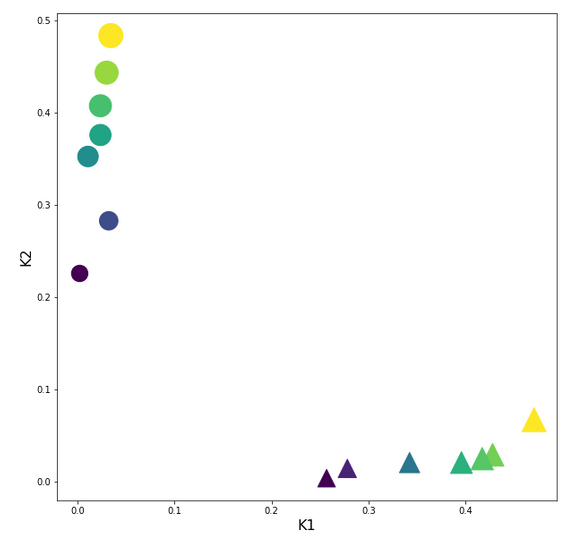Structurally very like the original; but with values reduced to [0,1]. This was to be expected.

# The cost hyperplane for the data normalized "per feature"

After the transformation of the sample data the cost hyperplane over the {w1, w2}-space looks as follows: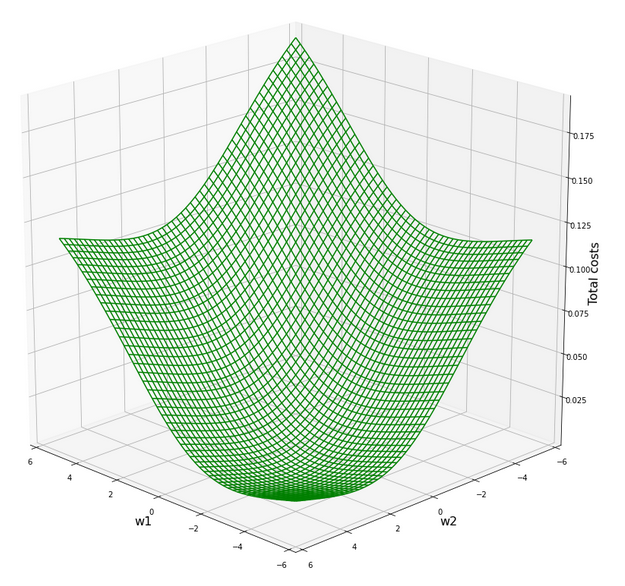We see a clear minimum; it does, however, not appear as pronounced as for the StandardScaler, which we applied in the last article.

But: There are no side valleys with small gradients at the end of the steep slope area. This means that a path into a minimum will probably look a bit different compared to a path on the hyperplane we got with the "StandardScaler".

Our mesh in the {w1, w2}-space indicates the following position of the minimum:

```C_min =  0.0006350159045771724
Coordinates:  [[3949 1542]]
Weight values at cost minimum: -2.4160000000003397 2.39799999999913
```

# Gradient descent results after normalization per feature with "Normalizer"

With our gradient descent method and the following run-parameters

w1_start = -0.20, w2_start = 0.25 eta = 0.2, decrease_rate = 0.00000001, num_steps = 2500

we get the following result of a run which explores both stochastic and batch gradient descent:

```Stoachastic Descent
Kt1       Kt2     K1     K2  Tgt       Res       Err
0   0.427867  0.029555  200.0   14.0  0.3  0.276365  0.078783
1   0.002139  0.225885    1.0  107.0  0.7  0.630971  0.098613
2   0.342294  0.021111  160.0   10.0  0.3  0.315156  0.050519
3   0.023533  0.407437   11.0  193.0  0.7  0.715038  0.021483
4   0.470654  0.067554  220.0   32.0  0.3  0.273924  0.086920
5   0.023533  0.375771   11.0  178.0  0.7  0.699320  0.000971
6   0.256720  0.004222  120.0    2.0  0.3  0.352075  0.173584
7   0.029951  0.443325   14.0  210.0  0.7  0.729191  0.041701
8   0.417171  0.025333  195.0   12.0  0.3  0.279519  0.068271
9   0.032090  0.282884   15.0  134.0  0.7  0.645816  0.077405
10  0.278114  0.014778  130.0    7.0  0.3  0.346085  0.153615
11  0.010697  0.352549    5.0  167.0  0.7  0.694107  0.008418
12  0.395777  0.021111  185.0   10.0  0.3  0.287962  0.040126
13  0.034229  0.483436   16.0  229.0  0.7  0.745803  0.065432

Batch Descent
Kt1       Kt2     K1     K2  Tgt       Res       Err
0   0.427867  0.029555  200.0   14.0  0.3  0.276360  0.078799
1   0.002139  0.225885    1.0  107.0  0.7  0.630976  0.098606
2   0.342294  0.021111  160.0   10.0  0.3  0.315152  0.050505
3   0.023533  0.407437   11.0  193.0  0.7  0.715045  0.021493
4   0.470654  0.067554  220.0   32.0  0.3  0.273919  0.086935
5   0.023533  0.375771   11.0  178.0  0.7  0.699326  0.000962
6   0.256720  0.004222  120.0    2.0  0.3  0.352072  0.173572
7   0.029951  0.443325   14.0  210.0  0.7  0.729198  0.041711
8   0.417171  0.025333  195.0   12.0  0.3  0.279514  0.068287
9   0.032090  0.282884   15.0  134.0  0.7  0.645821  0.077398
10  0.278114  0.014778  130.0    7.0  0.3  0.346081  0.153603
11  0.010697  0.352549    5.0  167.0  0.7  0.694113  0.008410
12  0.395777  0.021111  185.0   10.0  0.3  0.287957  0.040142
13  0.034229  0.483436   16.0  229.0  0.7  0.745810  0.065443

Total error stoch descent:  0.06898872490256348
Total error batch descent:  0.06899042421795792
```

Good! Seemingly we got some convergence in both cases. The overall "accuracy" achieved on the training set is even a bit better than for the "StandardScaler". And:

```Final (w1,w2)-values stoch : ( -2.4151 ,  2.3977 )
Final (w1,w2)-values batch : ( -2.4153 ,  2.3976 )
```

This fits very well to the data we got from our mesh analysis of the cost hyperplane!

Regarding the evolution of the costs and the weights we see a slightly different picture than with the "StandardScaler":

Cost and weight evolution during stochastic gradient descent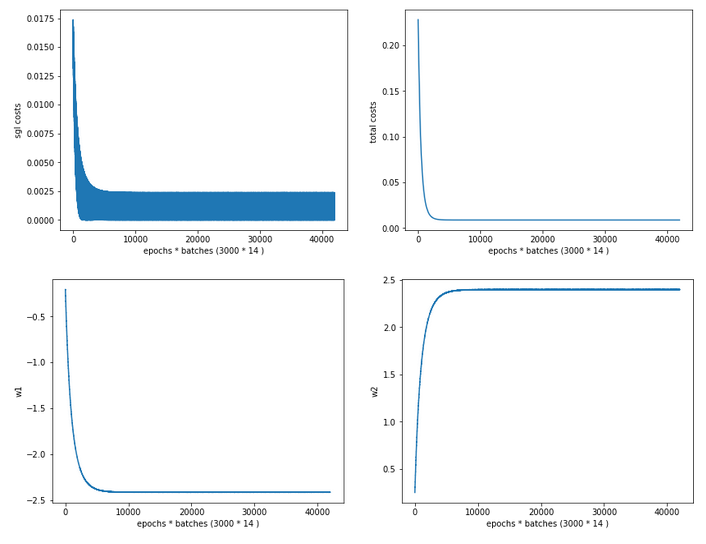and:

Cost and weight evolution during batch gradient descent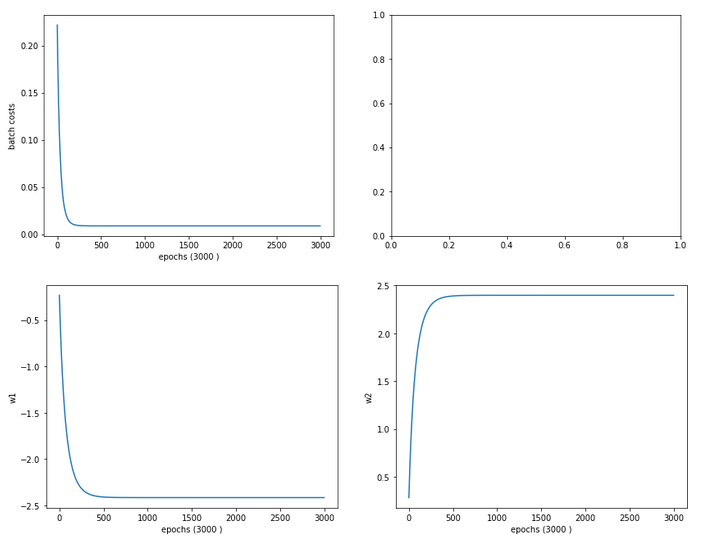From the evolution of the weight parameters we can assume that gradient descent moved along a direct path into the cost minimum. This fits to the different shape of the cost hyperplane in comparison with the hyperplane we got after the application of the "StandardScaler".

# Predicted contour and separation lines in the {K1, K2}-plane after feature-scaling with "Normalizer"

We compute the contour lines of the output A of our solitary neuron (see article 1 of this series) with the following code:

```
# ***********
# Contours
# ***********
from matplotlib import ticker, cm

# Take w1/w2-vals from above w1f, w2f
w1_len = len(li_w1_ba)
w2_len = len(li_w1_ba)
w1f = li_w1_ba[w1_len -1]
w2f = li_w2_ba[w2_len -1]

def A_mesh(w1,w2, Km1, Km2):
kshape = Km1.shape
A = np.zeros(kshape)

Km1V = Km1.reshape(kshape*kshape, )
Km2V = Km2.reshape(kshape*kshape, )
print("km1V.shape = ", Km1V.shape, "\nkm1V.shape = ", Km2V.shape )

# scaling trafo
if scale_method == 0:
Km1V = scaling_fact_K1 * Km1V
Km2V = scaling_fact_K2 * Km2V
KmV = np.vstack( (Km1V, Km2V) )
KmT = KmV.T
else:
KmV = np.column_stack((Km1V, Km2V))
KmT = scaler.transform(KmV)

Km1T, Km2T = KmT.T
Km1TR = Km1T.reshape(kshape)
Km2TR = Km2T.reshape(kshape)
print("km1TR.shape = ", Km1TR.shape, "\nkm2TR.shape = ", Km2TR.shape )

rg_idx = range(num_samples)
Z      = w1 * Km1TR + w2 * Km2TR
A = expit(Z)
return A

#Build K1/K2-mesh
minK1, maxK1 = li_K1.min()-20, li_K1.max()+20
minK2, maxK2 = li_K2.min()-20, li_K2.max()+20
resolution = 0.1
Km1, Km2 = np.meshgrid( np.arange(minK1, maxK1, resolution),
np.arange(minK2, maxK2, resolution))

A = A_mesh(w1f, w2f, Km1, Km2 )
print("A.shape = ", A.shape)

fig_size = plt.rcParams["figure.figsize"]
#print(fig_size)
fig_size = 14
fig_size = 11
fig, ax = plt.subplots()
cmap=cm.PuBu_r
cmap=cm.RdYlBu
#cs = plt.contourf(X, Y, Z1, levels=25, alpha=1.0, cmap=cm.PuBu_r)
cs = ax.contourf(Km1, Km2, A, levels=25, alpha=1.0, cmap=cmap)
cbar = fig.colorbar(cs)
N = 14
r0 = 0.6
x = li_K1
y = li_K2
area = 6*np.sqrt(x ** 2 + y ** 2)  # 0 to 10 point radii
c = np.sqrt(area)
r = np.sqrt(x ** 2 + y ** 2)
area1 = np.ma.masked_where(x < 100, area)
area2 = np.ma.masked_where(x >= 100, area)
ax.scatter(x, y, s=area1, marker='^', c=c)
ax.scatter(x, y, s=area2, marker='o', c=c)
# Show the boundary between the regions:
ax.set_xlabel("K1", fontsize=16)
ax.set_ylabel("K2", fontsize=16)

```

Please note the differences in how we handle the creation of the array "KmT" with the transformed data for "scale_method=0", i.e. "Normalizer", in comparison to other methods.

Here is the result: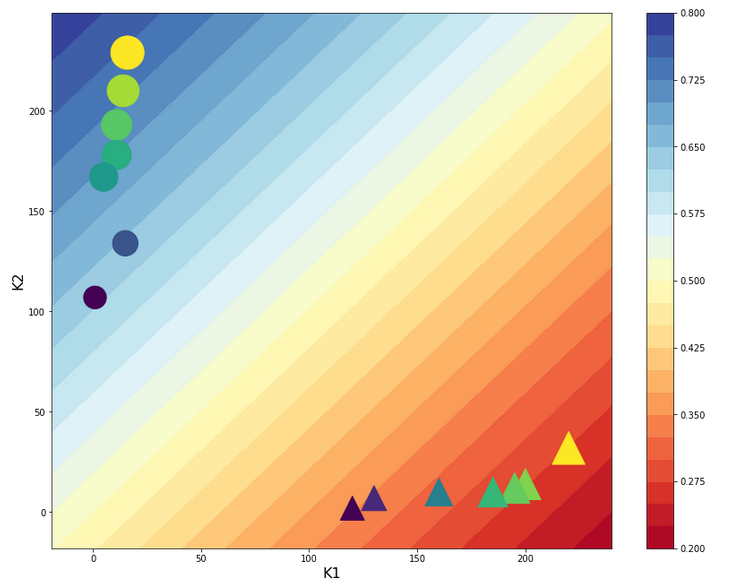Looks very similar to our plot for the StandardScaler in the last article - but with a slight shift on the K1-axis. So, the answer to our first question is: The contour lines are straight diagonal lines!

This is a direct result of the equations

expit(z) = E_z = const. => z = const. => w1*f1*K1 + w2*f2*K2 = C_z =>
K2 = C_k -fact*K1

The last one is nothing but an equation for a straight line. As "factor" is a constant, the angle α with the K1-axis remains the same for different E_z and C_k, i.e. we get parallel lines. If "fact = "-w1*f1/w2*f2 ≈ 1 = tan(α)" we get almost a 45°ree;-angle α. Let us see in our case : w1 = -2.4151 , w2 = 2.3977, f1 = 0.00214, f2 = 0.00211 => fact = 1.0215. This explains our plot.

# "Normalizer" used per sample

Now we scale the (K1, K2) coordinates in feature space of each single sample with the Normalizer. I.e. we scale K1 and K2 for each individual sample by a common factor 1/sqrt(K1**2 + K2**2). Meaning: No scaling with a common factor per feature over all samples; instead scaling of the features per sample. As already said: If we regard K1 and K2 as coordinates of a vector then we scale the distance of vectors end point radially to the origin of the coordinate system down to a length of 1.

Thus: After this normalization transformation we expect that our points are located on a unit circle! Note, however, that our transformation keeps up the angular distance of all data points. By "angular distance" for two selected points we mean the difference of the angles of these data points with e.g. the K1-axis.

Let us look at the transformed sample points in the {K1, K2}-plane:

Ok, our transformation has done a more pronounced "clustering" for us. Our transformed clusters are even more clearly separated from each other than before!

What does this mean for our cost hyperplane in the {w1, w2}-space? Well, here is a mesh-plot:

Cost hyperplane of the data scaled per sample by "Normalizer" in the {w1, w2}-space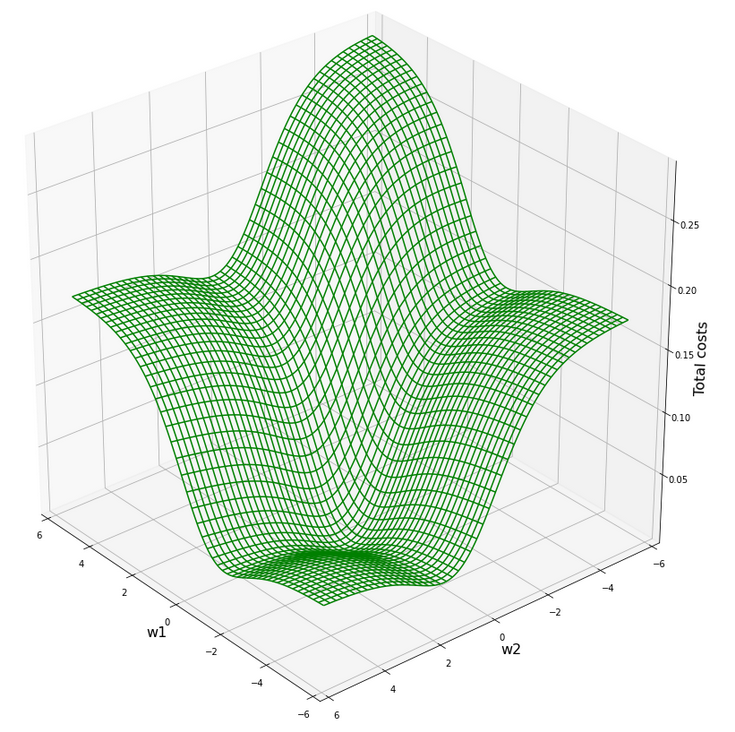According to our mesh the minimum is located at:

```C_min =  2.2726781812937556e-05
Coordinates:  [[3200 2296]]
Weight values at cost minimum: -0.9080000000005057 0.8999999999992951
```

# Comparison of the cost hyperplane with center of the original hyperplane for the unscaled batch data

Now comes a really funny point: Do you remember that we have gotten a similar plot before? Actually, we did when we looked at a tiny surroundings of the center of the cost hyperplane of the original unscaled data in the first article of this series:

Cost hyperplane at the center of the original unscaled input data in the {w1, w2}-space?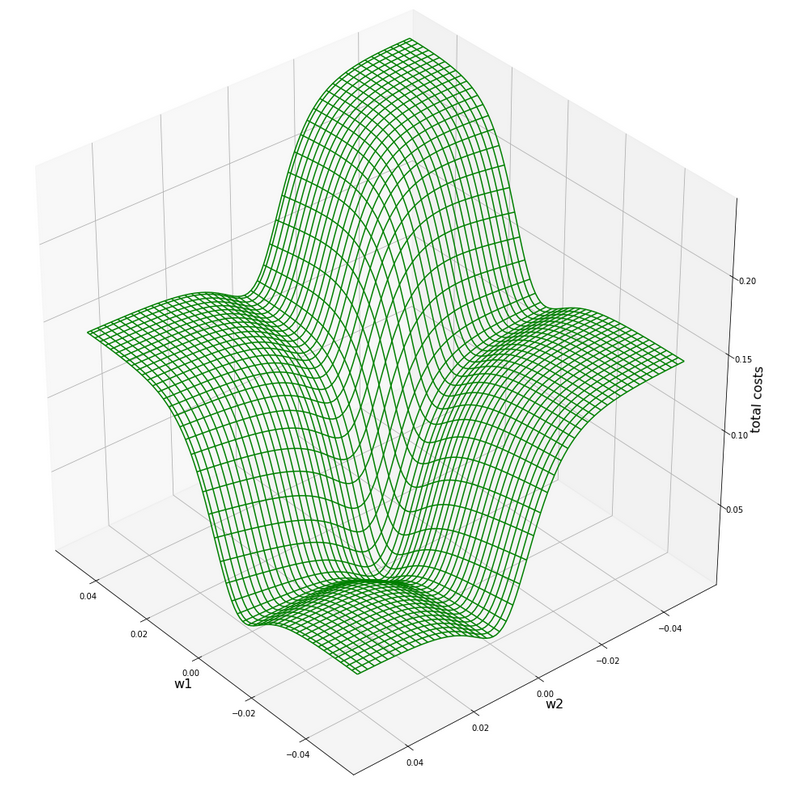A somewhat different viewing angle - but the similarity is obvious. Note however the very different scales of the (w1, w2)-values compared to the version of the scaled data.

How do we explain this similarity? Part of the answer lies in the fact that the total costs of the batch are dominated by those samples who have the biggest coordinate values, i.e. of those points where either K1 or K2 is biggest. Now, these points were very close to each other in the original data set. Now, for such points a centric stretch by a factor of around 1/200 would require a centric stretch (but now an expansion!) for the (w1, w2)-data with a reciprocate factor if we wanted to reproduce the same cost values. Reason: Linear coupling w1*K1+w2*K2! You compensate a constant factor in the {K1,K2}-space by its reciprocate one in the {w1, W2}-space!

But that is more or less what we have done by our somewhat strange application of the "Normalizer"! At least almost ... Fun, isn't it?

# Gradient descent after sample-wise (!) normalization by the "Normalizer"

The clearer separation of the clusters in the {K1, K2}-space after separation and a well formed cost hyperplane over the {w1, w2}-space should help us a bit with our gradient descent. We set the parameters of a gradient descent run to

w1_start = -0.20, w2_start = 0.25 eta = 0.2, decrease_rate = 0.00000001, num_steps = 1000

and get:

```Stoachastic Descent
Kt1       Kt2     K1     K2  Tgt       Res       Err
0   0.997559  0.069829  200.0   14.0  0.3  0.300715  0.002383
1   0.009345  0.999956    1.0  107.0  0.7  0.709386  0.013408
2   0.998053  0.062378  160.0   10.0  0.3  0.299211  0.002629
3   0.056902  0.998380   11.0  193.0  0.7  0.700095  0.000136
4   0.989586  0.143940  220.0   32.0  0.3  0.316505  0.055018
5   0.061680  0.998096   11.0  178.0  0.7  0.699129  0.001244
6   0.999861  0.016664  120.0    2.0  0.3  0.290309  0.032305
7   0.066519  0.997785   14.0  210.0  0.7  0.698144  0.002652
8   0.998112  0.061422  195.0   12.0  0.3  0.299019  0.003269
9   0.111245  0.993793   15.0  134.0  0.7  0.688737  0.016090
10  0.998553  0.053768  130.0    7.0  0.3  0.297492  0.008360
11  0.029927  0.999552    5.0  167.0  0.7  0.705438  0.007769
12  0.998542  0.053975  185.0   10.0  0.3  0.297533  0.008223
13  0.069699  0.997568   16.0  229.0  0.7  0.697493  0.003581

Batch Descent
Kt1       Kt2     K1     K2  Tgt       Res       Err
0   0.997559  0.069829  200.0   14.0  0.3  0.300723  0.002409
1   0.009345  0.999956    1.0  107.0  0.7  0.709388  0.013411
2   0.998053  0.062378  160.0   10.0  0.3  0.299219  0.002604
3   0.056902  0.998380   11.0  193.0  0.7  0.700097  0.000139
4   0.989586  0.143940  220.0   32.0  0.3  0.316513  0.055044
5   0.061680  0.998096   11.0  178.0  0.7  0.699131  0.001241
6   0.999861  0.016664  120.0    2.0  0.3  0.290316  0.032280
7   0.066519  0.997785   14.0  210.0  0.7  0.698146  0.002649
8   0.998112  0.061422  195.0   12.0  0.3  0.299027  0.003244
9   0.111245  0.993793   15.0  134.0  0.7  0.688739  0.016087
10  0.998553  0.053768  130.0    7.0  0.3  0.297500  0.008335
11  0.029927  0.999552    5.0  167.0  0.7  0.705440  0.007771
12  0.998542  0.053975  185.0   10.0  0.3  0.297541  0.008198
13  0.069699  0.997568   16.0  229.0  0.7  0.697495  0.003578

Total error stoch descent:  0.011219103621660675
Total error batch descent:  0.01121352661948904
```

Well, this is a almost perfect result on the training set; just between 1% and 3% deviation from the aspired output values. We have obviously found something new! Before, we always had deviations up to 15% or even 20% in the prediction for some of the data samples in our training set.

The final values of the weights become:

```Final (w1,w2)-values stoch : ( -0.9093 ,  0.9009 )
Final (w1,w2)-values batch : ( -0.9090 ,  0.9009 )
```

Also very perfect. You should not forget - we worked with just 14 samples and 1 neuron.

The evolution data look like:

Cost and weight evolution during stochastic gradient descentand:

Cost and weight evolution during batch gradient descent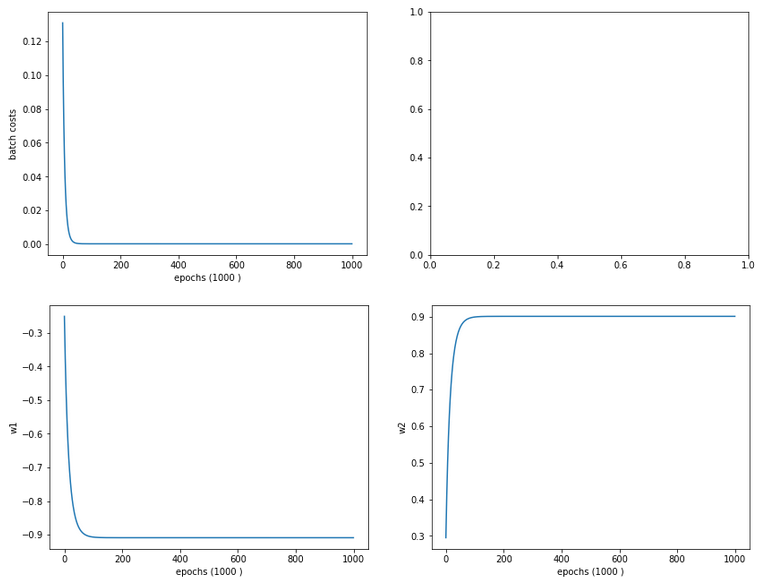Smooth development; fast convergence!

# Separation lines in the {K1, K2}-space after "per sample"-normalization with "Normalizer"

Now we turn to the answer to the second question we asked above: What changes regarding the separation or contour lines of the output values of our solitary neuron? Well as in our last article, we are interested in the output of our neuron after the normalization transformation of the data. I.e. we are on the search for contour lines, which we get for those points in the original {K1, K2}-space for which the sigmoid function produces a constant after transformation.

Here is the plot: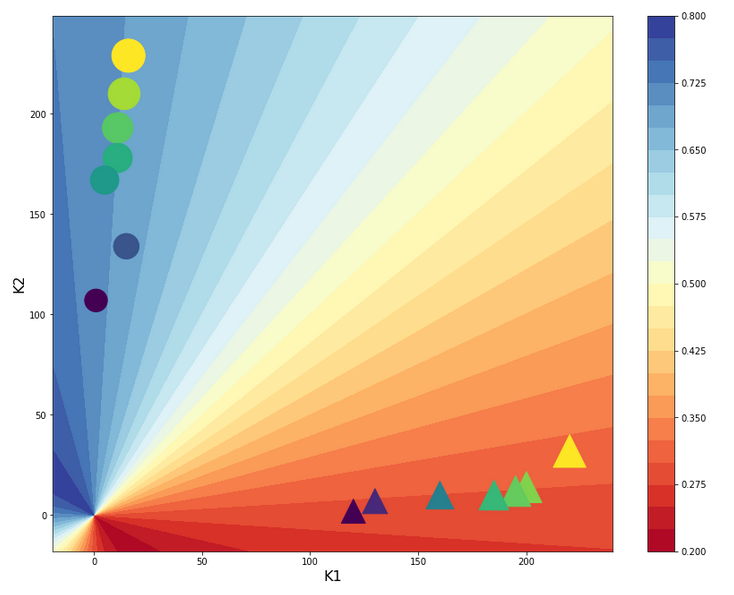Ooops, now we get a real difference. The contour curves are straight lines, but now directed radially outwards from the origin into the {K1, K2}-space! You see in addition that most of the data points are located very close to the lines for the set values A=0.3 and A=0.7!

We also get a very clear separation line close to diagonal at 45°ree;. A few comments on this finding:

The subdivision of the {K1, K2}-plane into sectors is very appropriate for clusters with data which show a tendency of a constant ration between the K1 and K2 values or clusters with a narrow extension in both directions. Note, however, that if we had two clusters at different radial distances but at roughly the same angle our present Normalizer transformation per sample would not have been helpful but disastrous regarding separation. So: The application of special normalization procedures ahead of classification training must be done with a feeling or insight into the clustering structure in the feature space.

What are the contour lines in the original {K1, K2}-space which produce the same output A for the transformed data? If we name the transformed (K1, K2) values by (k1, k2) we get in our case

k1 = K1/(K1**2 + K2**2)
k2 = K2/(K1**2 + K2**2)

So, we are looking for points in the {K1, K2}-space for which the equation

expit(w1*k1+ w2*k2) = const.

We now have to show that this is fulfilled for lines that have the property K2/K1 = tan(alpha) with alpha = const.. The proof is a small algebraic exercise, which I leave to my readers. Of course a genius like Mr Trump would give a direct answer based on the transformation properties itself: We just eliminated the radial distance to the origin as a feature! I leave it up to you which way of reasoning you want to go.

Our very specific way of using the "Normalizer" has led us to a clearer clustering after the scaling transformation. This gives rise to a fundamental idea:

What if you could use some method to detect clusters in the distribution of datapoints in feature space ahead of gradient decent?

But, on basis of what input or feature data then? Well, we could use some norm (as L2) to describe the distance of the data points from the centers of the different identified clusters as the new features! If we knew the centers of the clusters such an approach could have a potential advantage: It would set the the number of the new features to the number of the identified clusters. And this number could be substantially smaller than the number of originally features Why? Because in general not all features may be independent of each other and not all may be of major importance for the classification and cluster membership.

We shall follow this idea in my other series on a real MLP and MNIST in more detail.

# Conclusion

In this article we studied the application of the "Normalizer" offered by Scikit-Learn in two different ways to a training scenario for a one neuron perceptron and data with two input features (only). Normally we would apply "Normalizer" such that we would scale the data of all samples for each feature separately. And use the found stretching factors later on on new data points for which we want to make a classification prediction.

We saw that such a transformation roughly kept up the structure of the datapoint distribution in the {K1, K2}-fature-space. Scaling into an interval [-1, 1] had a major and healthy impact on the structure of the cost hyperplane in the {w1, w2}-weight-space. This helped us to perform a smooth gradient descent calculation.

Then we performed an application of "Normalizer" per sample. This corresponded to a radial stretch of all datapoints down to a unit cycle, whilst keeping up the values of the angles. We got a more structured cost hyperplane afterwards and a stronger clustering effect in the special case of our transformed data distribution in feature space. This helped gradient descent quite a lot: We could classify our data much better according to our discrimination prescription A=0.3 vs. A=0.7.

Our transformation also had the interesting effect of sub-dividing the feature space into radial sectors instead of parallel stripes. This would be helpful in case of data clusters with a certain radial elongation in the feature space but a clear difference and separation in angle. Such data do indeed exist - just think of the distribution of stars or microwave radiation clusters on the nightly sky sphere. At least in the latter case the radial distance of the sources may be of minor importance: You do not need radial distance information to note a concentration in a region which we call "milky way"!

What we actually did with our special normalization was to indirectly eliminate the radial distance information hidden in our (K1, K2)-data. We could also have calculated the angle (or a function of it) directly and thrown away all other information. If we had done so, we would have reduced our 2-dim the feature space to just one dimension! We saw this directly on the plot of the contour lines! Thus: It would have been much more intelligent, if we had used our transformation in a slightly modified form, determined just the angle of our data-points directly and uses these data as the only feature guiding gradient descent.

This led us to the idea that a clear identification of clusters by some appropriate method before we start a gradient descent analysis might be helpful for classification tasks.

This in turn triggers the idea of a cluster detection in feature space - which itself actually is a major discipline of Machine Learning. An advantage of using cluster detection ahead of gradient descent would be the possible reduction of the number of input features for the artificial neural network. Take a look at a forthcoming article in my other series on a Multilayer Perceptron [MLP] in this blog for an application in combination with a MLP and the MNIST daset.

In the next article of this series on a minimalistic perceptron we shall add a bias neuron to the input layer and investigate the impact.

# A single neuron perceptron with sigmoid activation function – II – normalization to overcome saturation

I continue my small series on a single neuron perceptron to study the positive effects of the normalization of input data in combination with the use of the sigmoid function as the activation function. In the last article

A single neuron perceptron with sigmoid activation function – I – failure of gradient descent due to saturation

we have seen that the saturation of the sigmoid function for big positive or negative arguments can prevent a smooth gradient descent under certain conditions - even if a global minimum clearly exists.

A perceptron with just one computing neuron is just a primitive example which demonstrates what can happen at the neurons of the first computing layer after the input layer of a real "Artificial Neural Network" [ANN]. We should really avoid to provide too big input values there and take into account that input values for different features get added up.

# Measures against saturation at neurons in the first computing layer

There are two elementary methods to avoid saturation of sigmoid like functions at neurons of the first hidden layer:

• Normalization: One measure to avoid big input values is to normalize the input data. Normalization can be understood as a transformation of given real input values for all of the features into an interval [0, 1] or [-1, 1]. There are of course many transformations which map a real number distribution into a given limited interval. Some keep up the relative distance of data points, some not. We shall have a look at some standard normalization variants used in Machine Learning [ML] during this and the next article .
The effect with respect to a sigmoidal activation function is that the gradient for arguments in the range [-1, 1] is relatively big. The sigmoid function behaves almost as a linear function in this argument region; see the plot in the last article.
• Choosing an appropriate (statistical) initial weight distribution: If we have a relatively big feature space as e.g. for the MNIST dataset with 784 features, normalization alone is not enough. The initial value distribution for weights must also be taken care of as we add up contributions of all input nodes (multiplied by the weights). We can follow a recommendation of LeCun (1990); see the book of Aurelien Geron recommended (here) for more details.
Then we would choose a uniform distribution of values in a range [-alpha*sqrt(1/num_inp_nodes), alpha*sqrt(1/num_inp_nodes)], with alpha \$asymp; 1.73 and num_inp_nodes giving the number of input nodes, which typically is the number of features plus 1, if you use a bias neuron. As a rule of thumb I personally take [-0.5*sqrt(1/num_inp_nodes, 0.5*sqrt[1/num_inp_nodes].

# Normalization functions

The following quick&dirty Python code for a Jupyter cell calls some normalization functions for our simple perceptron scenario and directly executes the transformation; I have provided the required import statements for libraries already in the last article.

```# ********
# Scaling
# ********

b_scale = True
scale_method = 3
# 0: Normalizer (standard), 1: StandardScaler, 2. By factor, 3: Normalizer per pair
# 4: Min_Max, 5: Identity (no transformation) - just there for convenience

shape_ay = (num_samples,)
ay_K1 = np.zeros(shape_ay)
ay_K2 = np.zeros(shape_ay)

# apply scaling
if b_scale:
# shape_input = (num_samples,2)
rg_idx = range(num_samples)
if scale_method == 0:
shape_input = (2, num_samples)
ay_K = np.zeros(shape_input)
for idx in rg_idx:
ay_K[idx] = li_K1[idx]
ay_K[idx] = li_K2[idx]
scaler = Normalizer()
ay_K = scaler.fit_transform(ay_K)
for idx in rg_idx:
ay_K1[idx] = ay_K[idx]
ay_K2[idx] = ay_K[idx]
print(ay_K1)
print("\n")
print(ay_K2)
elif scale_method == 1:
shape_input = (num_samples,2)
ay_K = np.zeros(shape_input)
for idx in rg_idx:
ay_K[idx] = li_K1[idx]
ay_K[idx] = li_K2[idx]
scaler = StandardScaler()
ay_K = scaler.fit_transform(ay_K)
for idx in rg_idx:
ay_K1[idx] = ay_K[idx]
ay_K2[idx] = ay_K[idx]
elif scale_method == 2:
dmax = max(li_K1.max() - li_K1.min(), li_K2.max() - li_K2.min())
ay_K1 = 1.0/dmax * li_K1
ay_K2 = 1.0/dmax * li_K2
elif scale_method == 3:
shape_input = (num_samples,2)
ay_K = np.zeros(shape_input)
for idx in rg_idx:
ay_K[idx] = li_K1[idx]
ay_K[idx] = li_K2[idx]
scaler = Normalizer()
ay_K = scaler.fit_transform(ay_K)
for idx in rg_idx:
ay_K1[idx] = ay_K[idx]
ay_K2[idx] = ay_K[idx]
elif scale_method == 4:
shape_input = (num_samples,2)
ay_K = np.zeros(shape_input)
for idx in rg_idx:
ay_K[idx] = li_K1[idx]
ay_K[idx] = li_K2[idx]
scaler = MinMaxScaler()
ay_K = scaler.fit_transform(ay_K)
for idx in rg_idx:
ay_K1[idx] = ay_K[idx]
ay_K2[idx] = ay_K[idx]
elif scale_method == 5:
ay_K1 = li_K1
ay_K2 = li_K2

# Get overview over costs on weight-mesh
wm1 = np.arange(-5.0,5.0,0.002)
wm2 = np.arange(-5.0,5.0,0.002)
#wm1 = np.arange(-0.3,0.3,0.002)
#wm2 = np.arange(-0.3,0.3,0.002)
W1, W2 = np.meshgrid(wm1, wm2)
C, li_C_sgl = costs_mesh(num_samples = num_samples, W1=W1, W2=W2, li_K1 = ay_K1, li_K2 = ay_K2, \
li_a_tgt = li_a_tgt)

C_min = np.amin(C)
print("C_min = ", C_min)
IDX = np.argwhere(C==C_min)
print ("Coordinates: ", IDX)
wmin1 = W1[IDX][IDX]
wmin2 = W2[IDX][IDX]
print("Weight values at cost minimum:",  wmin1, wmin2)

# Plots
# ******
fig_size = plt.rcParams["figure.figsize"]
#print(fig_size)
fig_size = 19; fig_size = 19

fig3 = plt.figure(3); fig4 = plt.figure(4)

ax3 = fig3.gca(projection='3d')
ax3.get_proj = lambda: np.dot(Axes3D.get_proj(ax3), np.diag([1.0, 1.0, 1, 1]))
ax3.view_init(25,135)
ax3.set_xlabel('w1', fontsize=16)
ax3.set_ylabel('w2', fontsize=16)
ax3.set_zlabel('Total costs', fontsize=16)
ax3.plot_wireframe(W1, W2, 1.2*C, colors=('green'))

ax4 = fig4.gca(projection='3d')
ax4.get_proj = lambda: np.dot(Axes3D.get_proj(ax4), np.diag([1.0, 1.0, 1, 1]))
ax4.view_init(25,135)
ax4.set_xlabel('w1', fontsize=16)
ax4.set_ylabel('w2', fontsize=16)
ax4.set_zlabel('Single costs', fontsize=16)
ax4.plot_wireframe(W1, W2, li_C_sgl, colors=('blue'))
#ax4.plot_wireframe(W1, W2, li_C_sgl, colors=('red'))
ax4.plot_wireframe(W1, W2, li_C_sgl, colors=('orange'))
#ax4.plot_wireframe(W1, W2, li_C_sgl, colors=('yellow'))
#ax4.plot_wireframe(W1, W2, li_C_sgl, colors=('magenta'))
#ax4.plot_wireframe(W1, W2, li_C_sgl, colors=('green'))

plt.show()

```

The results of the transformation for our two features are available in the arrays "ay_K1" and "ay_K2". These arrays will then be used as an input to gradient descent.

# Some remarks on some normalization methods:

Normalizer: It is in the above code called by setting "scale_method=0". The "Normalizer" with standard parameters scales by applying a division by an averaged L2-norm distance. However, its application is different from other SciKit-Learn scalers:
It normalizes over all data given in a sample. The dimensions beyond 1 are NOT interpreted as features which have to be normalizes separately - as e.g. the "StandardScaler" does. So, you have to be careful with index handling! This explains the different index-operation for "scale_method = 0" compared to other cases.

StandardScaler: Called by setting "scale_method=1". The StandardScaler accepts arrays of samples with columns for features. It scales all features separately. It subtracts the mean average of all feature values of all samples and divides afterwards by the standard deviation. It thus centers the value distribution with a mean value of zero and a variance of 1. Note however that it does not limit all transformed values to the interval [-1, 1].

MinMaxScaler: Called by setting "scale_method=4". The MinMaxScaler
works similar to the StandardScaler but subtracts the minimum and divides by the (max-min)-difference. It therefore does not center the distribution and does not set the variance to 1. However, it limits the transformed values to the interval [-1, 1].

Normalizer per sample: Called by setting "scale_method=3". This applies the Normalizer per sample! I.e., it scales in our case both the given feature values for one single by their mean and standard deviation. This may at first sound totally meaningless. But we shall see in the next article that it is not in case for our special set of 14 input samples.

Hint: For the rest of this article we shall only work with the StandardScaler.

# Input data transformed by the StandardScaler

The following plot shows the input clusters after a transformation with the "StandardScaler":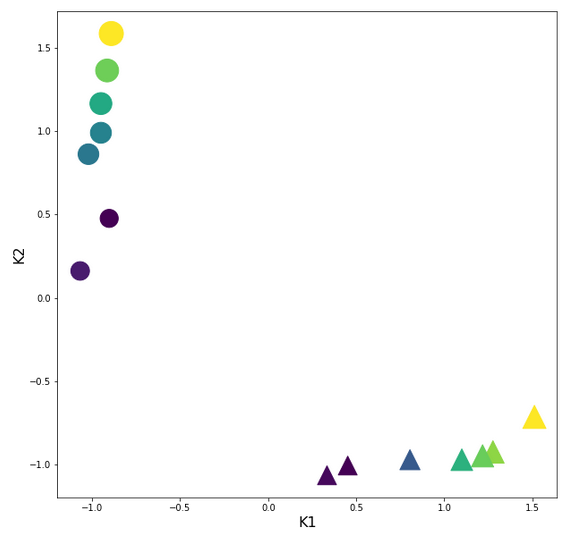You should recognize two things: The centralization of the features and the structural consistence of the clusters to the original distribution before scaling!

# The cost hyperplane over the {w1, w2}-space after the application of the StandardScaler to our input data

Let us apply the StandardScaler and look at the resulting cost hyperplane. When we set the parameters for a mesh display to

wm1 = np.arange(-5.0,5.0,0.002), wm2 = np.arange(-5.0,5.0,0.002)

we get the following results:

```C_min =  0.0006239618496774544
Coordinates:  [[2695 2259]]
Weight values at cost minimum: -0.4820000000004976 0.3899999999994064
```

Plots for total costs over the {w1, w2}-space from different angles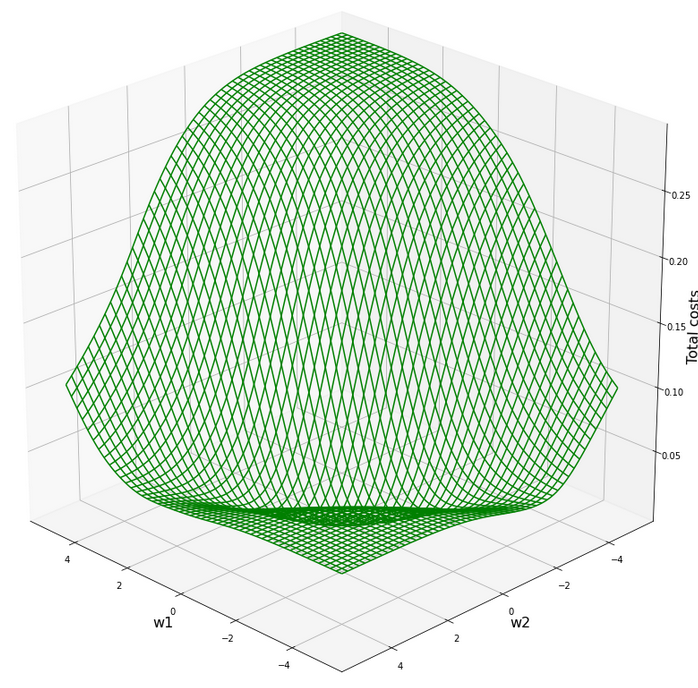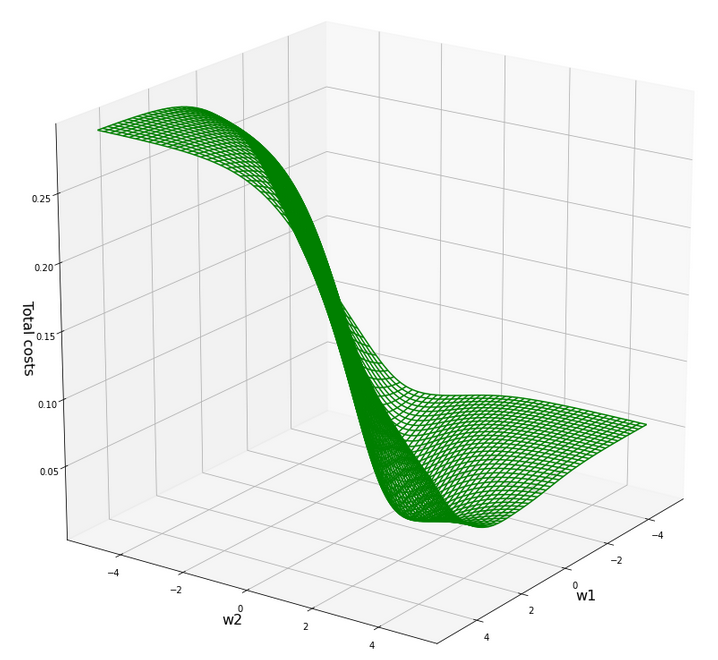Plot for individual costs (i=0, i=5) over the {w1, w2}-space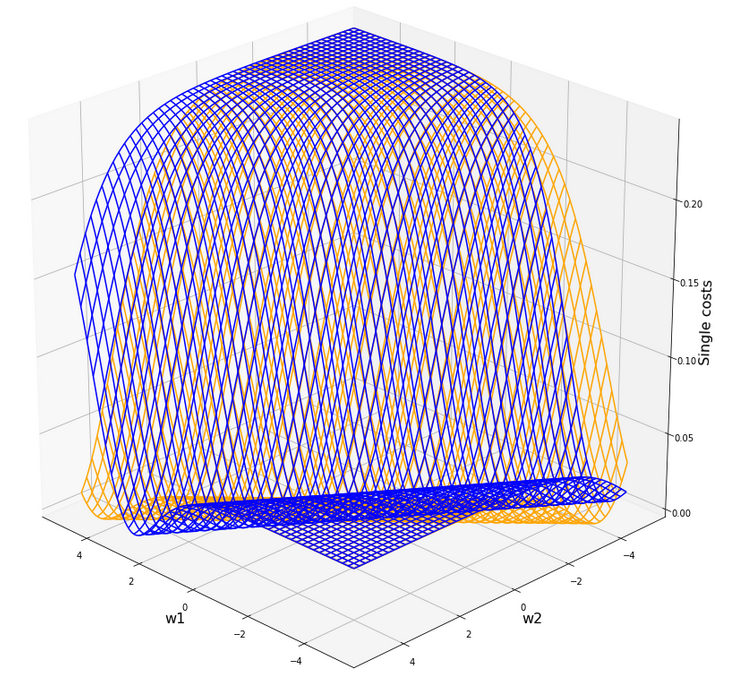The index "i" refers to our sample-array (see the last article).

# Gradient descent after scaling with the "StandardScaler"

Ok, let us now try gradient descent again. We set the following parameters:

w1_start = -0.20, w2_start = 0.25 eta = 0.1, decrease_rate = 0.000001, num_steps = 2000

Results:

```Stoachastic Descent
Kt1       Kt2     K1     K2  Tgt       Res       Err
0   1.276259 -0.924692  200.0   14.0  0.3  0.273761  0.087463
1  -1.067616  0.160925    1.0  107.0  0.7  0.640346  0.085220
2   0.805129 -0.971385  160.0   10.0  0.3  0.317122  0.057074
3  -0.949833  1.164828   11.0  193.0  0.7  0.713461  0.019230
4   1.511825 -0.714572  220.0   32.0  0.3  0.267573  0.108090
5  -0.949833  0.989729   11.0  178.0  0.7  0.699278  0.001031
6   0.333998 -1.064771  120.0    2.0  0.3  0.359699  0.198995
7  -0.914498  1.363274   14.0  210.0  0.7  0.725667  0.036666
8   1.217368 -0.948038  195.0   12.0  0.3  0.277602  0.074660
9  -0.902720  0.476104   15.0  134.0  0.7  0.650349  0.070930
10  0.451781 -1.006405  130.0    7.0  0.3  0.351926  0.173086
11 -1.020503  0.861322    5.0  167.0  0.7  0.695876  0.005891
12  1.099585 -0.971385  185.0   10.0  0.3  0.287246  0.042514
13 -0.890942  1.585067   16.0  229.0  0.7  0.740396  0.057709

Batch Descent
Kt1       Kt2     K1     K2  Tgt       Res       Err
0   1.276259 -0.924692  200.0   14.0  0.3  0.273755  0.087482
1  -1.067616  0.160925    1.0  107.0  0.7  0.640352  0.085212
2   0.805129 -0.971385  160.0   10.0  0.3  0.317118  0.057061
3  -0.949833  1.164828   11.0  193.0  0.7  0.713465  0.019236
4   1.511825 -0.714572  220.0   32.0  0.3  0.267566  0.108113
5  -0.949833  0.989729   11.0  178.0  0.7  0.699283  0.001025
6   0.333998 -1.064771  120.0    2.0  0.3  0.359697  0.198990
7  -0.914498  1.363274   14.0  210.0  0.7  0.725670  0.036672
8   1.217368 -0.948038  195.0   12.0  0.3  0.277597  0.074678
9  -0.902720  0.476104   15.0  134.0  0.7  0.650354  0.070923
10  0.451781 -1.006405  130.0    7.0  0.3  0.351924  0.173080
11 -1.020503  0.861322    5.0  167.0  0.7  0.695881  0.005884
12  1.099585 -0.971385  185.0   10.0  0.3  0.287241  0.042531
13 -0.890942  1.585067   16.0  229.0  0.7  0.740400  0.057714

Total error stoch descent:  0.07275422919538276
Total error batch descent:  0.07275715820661666
```

The attentive reader has noticed that I extended my code to include the columns with the original (K1, K2)-values into the Pandas dataframe. The code of the new function "predict_batch()" is given below. Do not forget to change the function calls at the end of the gradient descent code, too.

Now we obviously can speak of a result! The calculated (w1, w2)-data are:

```Final (w1,w2)-values stoch : ( -0.4816 ,  0.3908 )
Final (w1,w2)-values batch : ( -0.4815 ,  0.3906 )
```

Yeah, this is pretty close to the values we got via the fine grained mesh analysis of the cost function before! And within the error range!

Changed code for two of our functions in the last article

```def predict_batch(num_samples, w1, w2, ay_k_1, ay_k_2, li_K1, li_K2, li_a_tgt):
shape_res = (num_samples, 7)
ResData = np.zeros(shape_res)
rg_idx = range(num_samples)
err = 0.0
for idx in rg_idx:
z_in  = w1 * ay_k_1[idx] + w2 * ay_k_2[idx]
a_out = expit(z_in)
a_tgt = li_a_tgt[idx]
err_idx = np.absolute(a_out - a_tgt) / a_tgt
err += err_idx
ResData[idx] = ay_k_1[idx]
ResData[idx] = ay_k_2[idx]
ResData[idx] = li_K1[idx]
ResData[idx] = li_K2[idx]
ResData[idx] = a_tgt
ResData[idx] = a_out
ResData[idx] = err_idx
err /= float(num_samples)
return err, ResData

def create_df(ResData):
''' ResData: Array with result values K1, K2, Tgt, A, rel.err
'''
cols=["Kt1", "Kt2", "K1", "K2", "Tgt", "Res", "Err"]
df = pd.DataFrame(ResData, columns=cols)
return df
```

# How does the epoch evolution after the application of the StandardScaler look like?

Let us plot the evolution for the stochastic gradient descent:

Cost and weight evolution during stochastic gradient descent

Ok, we see that despite convergence the difference in the costs for different samples cannot be eliminated. It should be clear to the reader, why, and that this was to be expected.

We also see that the total costs (calculated from the individual costs) seemingly converges much faster than the weight values! Our gradient descent path obviously follows a big slope into a rather flat valley first (see the plot of the total costs above). Afterwards there is a small gradient sideways and down into the real minimum - and it obviously takes some epochs to get there. We also understand that we have to keep up a significant "learning rate" to follow the gradient in the flat valley. In addition the following rule seems to be appropriate sometimes:

We must not only watch the cost evolution but also the weight evolution - to avoid stopping gradient descent too early!

We shall keep this in mind for experiments with real multi-layer "Artificial Neural Networks" later on!

And how does the gradient descent based on the full "batch" of 14 samples look like?

Cost and weight evolution during batch gradient descent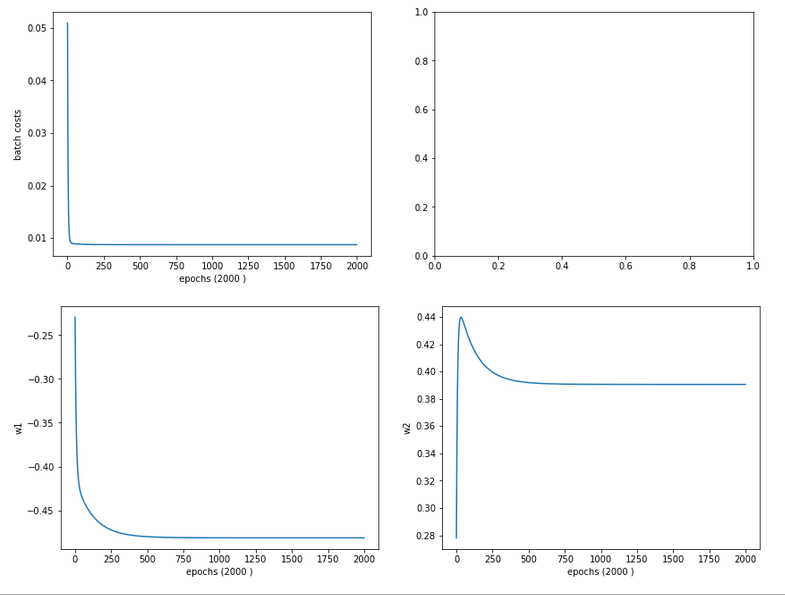A smooth beauty!

# Contour plot for separation curves in the {K1, K2}-plane

We add the following code to our Jupyter notebook:

```# ***********
# Contours
# ***********

from matplotlib import ticker, cm

# Take w1/w2-vals from above w1f, w2f
w1_len = len(li_w1_ba)
w2_len = len(li_w1_ba)
w1f = li_w1_ba[w1_len -1]
w2f = li_w2_ba[w2_len -1]

def A_mesh(w1,w2, Km1, Km2):
kshape = Km1.shape
A = np.zeros(kshape)

Km1V = Km1.reshape(kshape*kshape, )
Km2V = Km2.reshape(kshape*kshape, )
# print("km1V.shape = ", Km1V.shape, "\nkm1V.shape = ", Km2V.shape )

KmV = np.column_stack((Km1V, Km2V))

# scaling trafo
KmT = scaler.transform(KmV)

Km1T, Km2T = KmT.T
Km1TR = Km1T.reshape(kshape)
Km2TR = Km2T.reshape(kshape)
#print("km1TR.shape = ", Km1TR.shape, "\nkm2TR.shape = ", Km2TR.shape )

rg_idx = range(num_samples)
Z      = w1 * Km1TR + w2 * Km2TR
A = expit(Z)
return A

#Build K1/K2-mesh
minK1, maxK1 = li_K1.min()-20, li_K1.max()+20
minK2, maxK2 = li_K2.min()-20, li_K2.max()+20
resolution = 0.1
Km1, Km2 = np.meshgrid( np.arange(minK1, maxK1, resolution),
np.arange(minK2, maxK2, resolution))

A = A_mesh(w1f, w2f, Km1, Km2 )

fig_size = plt.rcParams["figure.figsize"]
fig_size = 14
fig_size = 11
fig, ax = plt.subplots()
cmap=cm.PuBu_r
cmap=cm.RdYlBu
#cs = plt.contourf(X, Y, Z1, levels=25, alpha=1.0, cmap=cm.PuBu_r)
cs = ax.contourf(Km1, Km2, A, levels=25, alpha=1.0, cmap=cmap)
cbar = fig.colorbar(cs)
N = 14
r0 = 0.6
x = li_K1
y = li_K2
area = 6*np.sqrt(x ** 2 + y ** 2)  # 0 to 10 point radii
c = np.sqrt(area)
r = np.sqrt(x ** 2 + y ** 2)
area1 = np.ma.masked_where(x < 100, area)
area2 = np.ma.masked_where(x >= 100, area)
ax.scatter(x, y, s=area1, marker='^', c=c)
ax.scatter(x, y, s=area2, marker='o', c=c)
# Show the boundary between the regions:
ax.set_xlabel("K1", fontsize=16)
ax.set_ylabel("K2", fontsize=16)

```

This code enables us to plot contours of predicted output values of our solitary neuron, i.e. A-values, on a mesh of the original {K1, K2}-plane. As we classified after a transformation of our input data, the following hint should be obvious:

Important hint: Of course you have to apply your scaling method to all the new input data created by the mesh-function! This is done in the above code in the "A_mesh()"-function with the following lines:

```    # scaling trafo
if (scale_method == 3):
KmT = scaler.fit_transform(KmV)
else:
KmT = scaler.transform(KmV)
```

We can directly apply the StandardScaler on our new data via its method transform(); the scaler will use the parameters it found during his first "scaler.fit_transform()"-operation on our input samples. However, we cannot do it this way when using the Normalizer for each individual new data sample via "scale_method =3". I shall come back to this point in a later article.

The careful reader also sees that our code will, for the time being, not work for scale_method=0, scale_method=2 and scale_method=5. Reason: I was too lazy to write a class or code suitable for these normalizing operations. I shall correct this when we need it.

But at least I added our input samples via scatter plotting to the final output. The result is: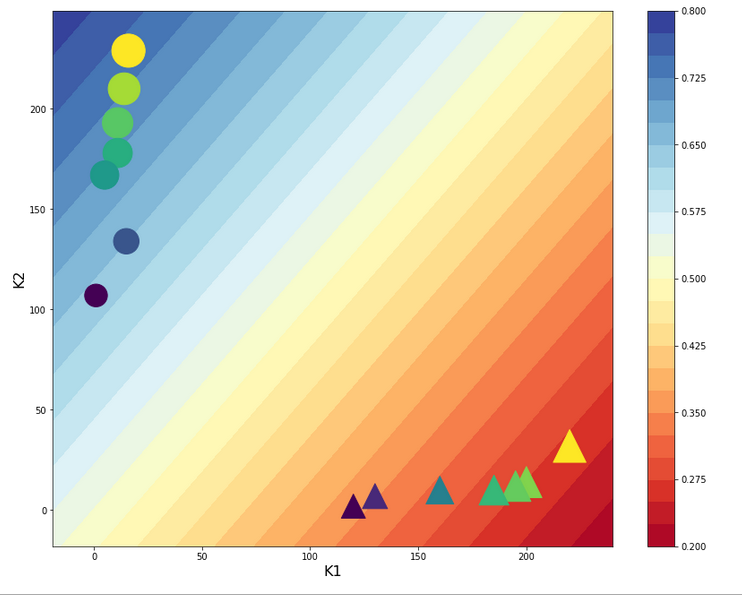The deviations from our target values is to be expected. With a given pair of (w1, w2)-values we cannot do much better with a single neuron and a linear weight impact on the input data.

But we see: If we set up a criterion like:

• A > 0.5 => sample belongs to the left cluster,
• A ≤ 0.5 => sample belongs to the right cluster

we would have a relatively good classificator available - based on one neuron only!

# Intermediate Conclusion

In this article I have shown that the "standardization" of input data, which are fed into a perceptron ahead of a gradient descent calculation, helps to circumvent problems with the saturation of the sigmoid function at the computing neuron following the input layer. We achieved this by applying the "StandardScaler" of Scikit-Learn. We got a smooth development of both the cost function and the weight parameters during gradient descent in the transformed data space.

We also learned another important thing:

An apparent convergence of the cost function in the vicinity of a minimum value does not always mean that we have reached the global minimum, yet. The evolution of the weight parameters may not yet have come to an end! Therefore, it is important to watch both the evolution of the costs AND the evolution of the weights during gradient descent. A too fast decline of the learning rate may not be good either under certain conditions.

In the next article

A single neuron perceptron with sigmoid activation function – III – two ways of applying Normalizer

we shall look at two other normalization methods for our simplistic scenario. One of them will give us an even better classificator.

Stay tuned and remain healthy ...

And Mr Trump:
One neuron can obviously learn something about the difference of big and small numbers. This leads me to two questions, which you as a "natural talent" on epidemics can certainly answer: How many neurons are necessary to understand something about an exponential epidemic development? And why did it take so much time to activate them?

# A single neuron perceptron with sigmoid activation function – I – failure of gradient descent due to saturation

Readers who follow my series on a Python program for a "Multilayer Perceptron" [MLP] have noticed that I emphasized the importance of a normalization of input data in my last article:

A simple Python program for an ANN to cover the MNIST dataset – XII – accuracy evolution, learning rate, normalization

Our MLP "learned" by searching for the global minimum of the loss function via the "gradient descent" method. Normalization seemed to improve a smooth path to convergence whilst our algorithm moved into the direction of a global minimum on the surface of the loss or cost functions hyperplane over the weight parameter space. At least in our case where we used the sigmoid function as the activation function of the artificial neurons. I indicated that the reason for our observations had to do with properties of this function - especially for neurons in the first hidden layer of an MLP.

In case of the 4-layer MLP, which I used on the MNIST dataset, I applied a special form of normalization namely "standardization". I did this by using the StandarScaler of SciKit-Learn. See the following link for a description: Sklearn preprocessing StandardScaler

We saw the smoothing and helpful impact of normalization on the general convergence of gradient descent by the help of a few numerical experiments. The interaction of the normalization of 784 features with mini-batches and with a complicated 4 layer-MLP structure, which requires the determination of several hundreds of thousands weight values is however difficult to grasp or analyze. One understands that there is a basic relation to the properties of the activation function, but the sheer number of the dimensions of the feature and weight spaces and statistics make a thorough understanding difficult.

Since then I have thought a bit about how to set up a really small and comprehensible experiment which makes the positive impact of normalization visible in a direct and visual form. I have come up with the following most simple scenario, namely the training of a simple perceptron with only one computing neuron and two additional "stupid" neurons in an input layer which just feed input data to our computing neuron: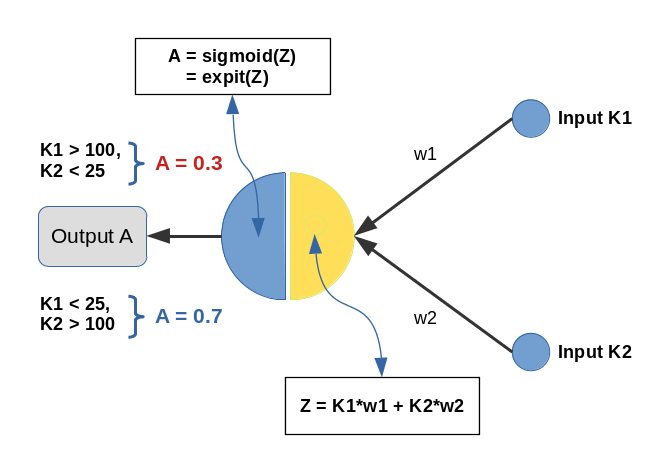Input values K1 and K2 are multiplied by weights w1 and w2 and added by the central solitary "neuron". We use the sigmoid function as the "activation function" of this neuron - well anticipating that the properties of this function may lead to trouble.

The perceptron has only one task: Discriminate between two different types of input data by assigning them two distinct output values.

• For K1 > 100 and K2 < 25 we want an output of A=0.3.
• For K1 &l; 25 and K2 > 100 we, instead, want an output of A=0.7

We shall feed the perceptron only 14 different pairs of input values K1[i], K2[i] (i =0,1,..13), e.g. in form of lists:

```li_K1 = [200.0,   1.0, 160.0,  11.0, 220.0,  11.0, 120.0,  22.0, 195.0,  15.0, 130.0,   5.0, 185.0,  16.0]
li_K2 = [ 14.0, 107.0,  10.0, 193.0,  32.0, 178.0,   2.0, 210.0,  12.0, 134.0,  15.0, 167.0,  10.0, 229.0]
```

(The careful reader detects one dirty example li_K2 = 32 (> 25), in contrast to our setting. Just to see how much of an impact such a deviation has ...)

We call each pair (K1, K2)=(li_K1[i], li_K2[i]) for a give "i" a "sample". Each sample contains values for two "features": K1 and K2. So, our solitary computing neuron has to solve a typical classification problem - it shall distinguish between two groups of input samples. In a way it must learn the difference between small and big numbers for 2 situations appearing at its input channels.

Off topic: This morning I listened to a series of comments of Mr. Trump during the last weeks on the development of the Corona virus crisis in the USA. Afterwards, I decided to dedicate this mini-series of articles on a perceptron to him - a person who claims to "maybe" be "natural talent" on complicated things as epidemic developments. Enjoy (?) his own words via an audio compilation in the following news article:
https://www.faz.net/aktuell/politik/trumps-praesidentschaft/usa-zehn-wochen-corona-in-den-worten-von-trump-16708603.html

# Two well separable clusters

In the 2-dim feature space {K1, K2} we have just two clusters of input data: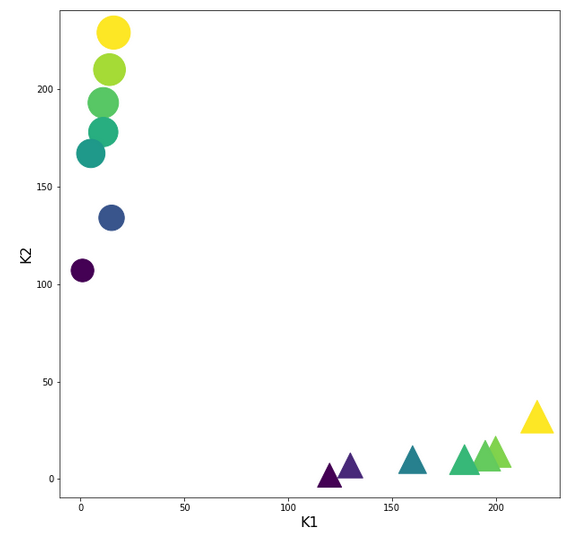Each cluster has a long diameter along one of the feature axes - but overall the clusters are well separable. A rather good separation surface would e.g. be a diagonal line.

For a given input sample with K-values K1 and K2 we define the output of our computing neuron to be

A(w1, w2) = expit( w1*K1 + w2*K2 ) ,

where expit() symbolizes the sigmoid function. See the next section for more details.

Corresponding target-values for the output A are (with at1 = 0.3 and at2 = 0.7):

```li_a_tgt = [at1,  at2,  at1,  at2,  at1,  at2,  at1,  at2,  at1,  at2,  at1,  at2,   at1,  at2]
```

With the help of these target values our poor neuron shall learn from the 14 input samples to which cluster a given future sample probably belongs to. We shall use the "gradient descent" method to train the neuron for this classification task. o solve the task our neuron must find a reasonable separation surface - a line - in the {K1,K2}-plane; but it got the additional task to associate two distinct output values "A" with the two clusters:

A=0.7 for data points with a large K1-value and A=0.3 for data points with a small K1-value.

So, the separation surface has to fulfill some side conditions.

Readers with a background in Machine Learning and MLPs will now ask: Why did you pick the special values 0.3 and 0.7? A good question - I will come back to it during our experiments. Another even more critical question could be: What about a bias neuron in the input layer? Don't we need it? Again, a very good question! A bias neuron allows for a shift of a separation surface in the feature space. But due to the almost symmetrical nature of our input data (see the positions and orientations of the clusters!) and the target values the impact of a bias neuron on the end result would probably only be small - but we shall come back to the topic of a bias neuron in a later article. But you are free to extend the codes given below to account for a bias neuron in the input layer. You will notice a significant impact if you change either the relative symmetry of the input or of the output data. But lets keep things simple for the next hours ...

# The sigmoid function and its saturation for big arguments

You can import the sigmoid function under the name "expit" from the "scipy" library into your Python code. The sigmoid function is a smooth one - but it quickly saturates for big negative or positive values: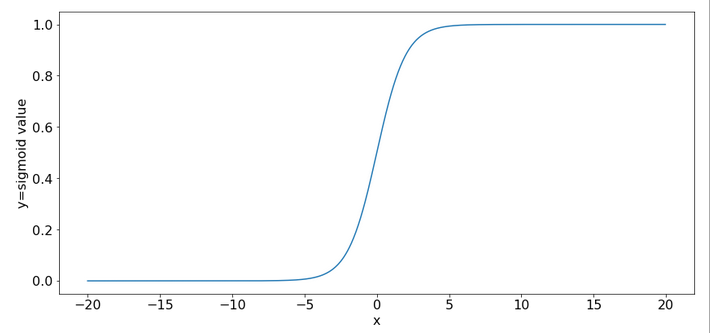So, output values get almost indistinguishable if the absolute values of the arguments are bigger than 15.

# What is interesting about our input data? What is the relation to MNIST data?

The special situation about the features in our example is the following: For one and the same feature we have a big number and a small number to work with - depending on the sample. Which feature value - K1 or K2 - is big depends on the sample.

This is something that also happens with the input "features" (i.e. pixels) coming from a MNIST-image:
For a certain feature (= a pixel at a defined position in the 28x28 picture) in a given sample (= a distinct image) we may get a big number as 255. For another feature (= pixel) the situation may be totally different and we may find a value of 0. In another sample (= picture) we may get the reverse situation for the same two pixels.

What happens in such a situation at a specific neuron in the first hidden neuron layer of a MLP when we start gradient descent with a statistical distribution of weight values? If we are unlucky then the initial statistical weight constellation for a sample may lead to a big number of the total input to our selected hidden neuron with the effect of a very small gradient at this node - due to saturation of the sigmoid function.

To give you a feeling: Assume that you have statistical weights in the range of [-0.025, 0.025]. Assume further that only 4 pixels of a MNIST picture with a value of 200 contribute with a local maximum weight of 0.25; then we get a a minimum input at our node of 4*0.25*200 = 20. The gradient of expit(20) has a value of 2e-9. Even if we multiply by the required factor of 200 for a weight correction at one of the contributing input nodes we would would arrive at 4e-7. Quite hopeless. Of course, the situation is not that bad for all weights and image-samples, but you get an idea about the basic problem ....

Our simple scenario breaks the MNIST situation down to just two features and just one working neuron - and therefore makes the correction situation for gradient descent really extreme - but interesting, too. And we can analyze the situation far better than for a MLP because we deal with an only 2-dimensional feature-space {K1, K2} and a 2-dimensional weight-space {w1, w2}.

# A simple quadratic cost function for our neuron

For given values w1 and w2, i.e. a tuple (w1, w2), we define a quadratic cost or loss function C_sgl for one single input sample (K1, K2) as follows:

C_sgl = 0.5 * ( li_a_tgt(i) - expit(z_i) )**2, with z_i = li_K[i]*w1 + li_K2[i]*w2

The total cost-function for the batch of all 14 samples is just the sum of all these terms for the individual samples.

# Existence of a solution for our posed problem?

From all we theoretically know about the properties of a simple perceptron it should be able to find a reasonable solution! But, how do we know that a reasonable solution for a (w1, w2)-pair does exist at all? One line of reasoning goes like follows:

For two samples - each a member of either cluster - you can plot the hyperplanes of the related outputs "A(K1, K2) = expit(w1*K1+w2*K2)" over the (w1, w2)-space. These hyperplanes are almost orthogonal to each other. If you project the curves of a cut with the A=0.3-planes and the A=0.7-planes down to the (w1, w2)-plane at the center you get straight lines - almost orthogonally oriented against each other. So, such 2 specific lines cut each other in a well defined point - somewhere off the center. As the expit()-function is a relatively steep one for our big input values the crossing point is relatively close to the center. If we choose other samples we would get slightly different lines and different crossing points of the projected lines - but not too far from each other.

The next plot shows the expit()-functions close to the center of the (w1, w2)-plane for two specific samples of either cluster. We have in addition displayed the surfaces for A=0.7 and A=0.3.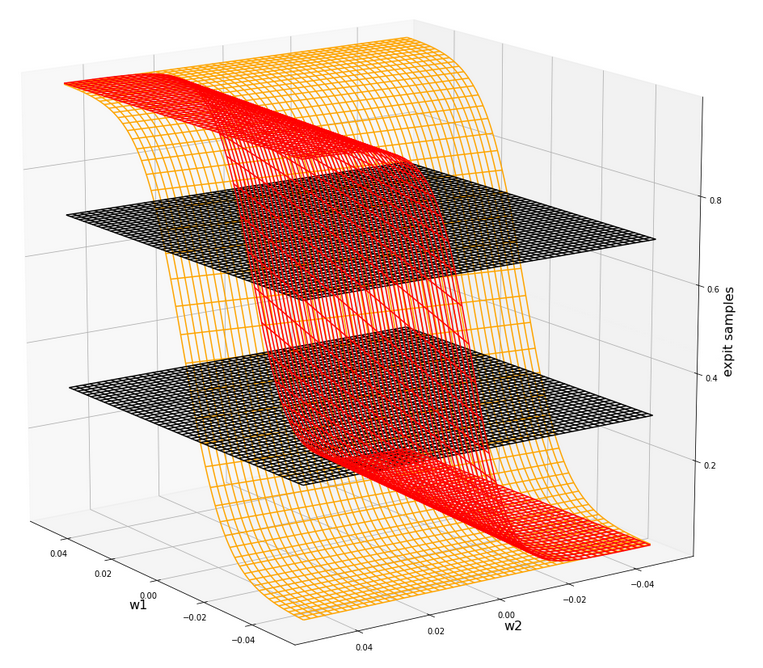The following plot shows the projections of the cuts of the surfaces for 7 samples of each cluster with the A=0.3-plane and the A=0.7, respectively.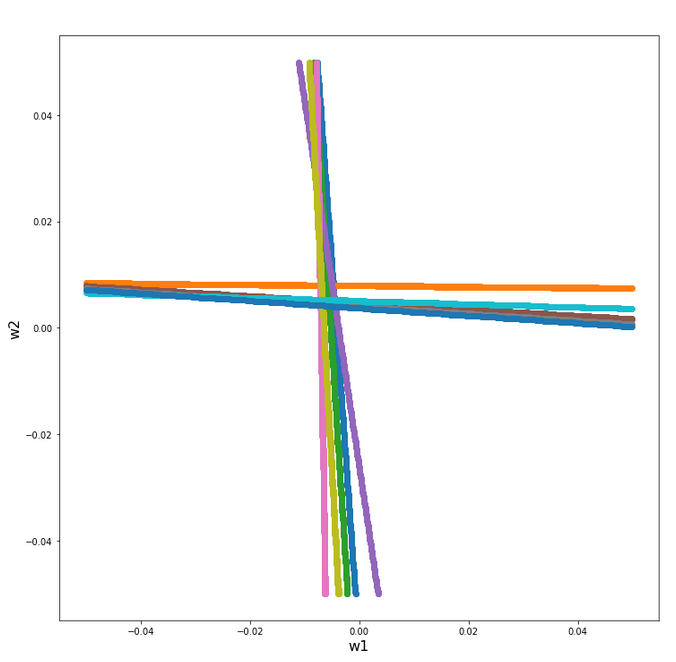The area of crossings is not too big on the chosen scale of w1, w2. Looking at the graphics we would expect an optimal point around (w1=-0.005, w2=+0.005) - for the original, unnormalized input data.

By the way: There is also a solution for at1=0.3 and at2=0.3, but a strange one. Such a setup would not allow for discrimination. We expect a rather strange behavior then. A first guess could be: The resulting separation curve in the (K1, K2)-plane would move out of the area between the two clusters.

# Code for a mesh based display of the costs over the weight-parameter space

Below you find the code suited for a Jupyter cell to get a mesh display of the cost values

```import numpy as np
import numpy as np
import random
import math
import sys

from sklearn.preprocessing import StandardScaler
from sklearn.preprocessing import Normalizer
from sklearn.preprocessing import MinMaxScaler
from scipy.special import expit

import matplotlib as mpl
from matplotlib import pyplot as plt
from matplotlib.colors import ListedColormap
import matplotlib.patches as mpat
from mpl_toolkits import mplot3d
from mpl_toolkits.mplot3d import Axes3D

# total cost functions for overview
# *********************************
def costs_mesh(num_samples, W1, W2, li_K1, li_K2, li_a_tgt):
zshape = W1.shape
li_Z_sgl = []
li_C_sgl = []
C = np.zeros(zshape)

rg_idx = range(num_samples)
for idx in rg_idx:
Z_idx      = W1 * li_K1[idx] + W2 * li_K2[idx]
A_tgt_idx  = li_a_tgt[idx] * np.ones(zshape)
C_idx = 0.5 * ( A_tgt_idx - expit(Z_idx) )**2
li_C_sgl.append( C_idx )
C += C_idx

C /= np.float(num_samples)
return C, li_C_sgl

# ******************
# Simple Perceptron
#*******************

# input at 2 nodes => 2 features K1 and K2 => there will be just one output neuron
li_K1 = [200.0,   1.0, 160.0,  11.0, 220.0,  11.0, 120.0,  14.0, 195.0,  15.0, 130.0,   5.0, 185.0,  16.0  ]
li_K2 = [ 14.0, 107.0,  10.0, 193.0,  32.0, 178.0,   2.0, 210.0,  12.0, 134.0,  7.0, 167.0,  10.0, 229.0 ]

# target values
at1 = 0.3; at2 = 0.7
li_a_tgt  = [at1,  at2,  at1,  at2,   at1,   at2,  at1,   at2,  at1,  at2,  at1,  at2,   at1,  at2 ]

# Change to np floats
li_K1 = np.array(li_K1)
li_K2 = np.array(li_K2)
li_a_tgt = np.array(li_a_tgt)

num_samples = len(li_K1)

# Get overview over costs on mesh
# *******************************
# Mesh of weight values
wm1 = np.arange(-0.2,0.4,0.002)
wm2 = np.arange(-0.2,0.2,0.002)
W1, W2 = np.meshgrid(wm1, wm2)
# costs
C, li_C_sgl  = costs_mesh(num_samples=num_samples, W1=W1, W2=W2, \
li_K1=li_K1, li_K2=li_K2, li_a_tgt = li_a_tgt)

# Mesh-Plots
# ********
fig_size = plt.rcParams["figure.figsize"]
print(fig_size)
fig_size = 19
fig_size = 19

fig1 = plt.figure(1)
fig2 = plt.figure(2)

ax1 = fig1.gca(projection='3d')
ax1.get_proj = lambda: np.dot(Axes3D.get_proj(ax1), np.diag([1.0, 1.0, 1, 1]))
ax1.view_init(15,148)
ax1.set_xlabel('w1', fontsize=16)
ax1.set_ylabel('w2', fontsize=16)
ax1.set_zlabel('single costs', fontsize=16)

#ax1.plot_wireframe(W1, W2, li_C_sgl, colors=('blue'))
#ax1.plot_wireframe(W1, W2, li_C_sgl, colors=('orange'))
ax1.plot_wireframe(W1, W2, li_C_sgl, colors=('orange'))
ax1.plot_wireframe(W1, W2, li_C_sgl, colors=('green'))
#ax1.plot_wireframe(W1, W2, li_C_sgl, colors=('orange'))
#ax1.plot_wireframe(W1, W2, li_C_sgl, colors=('magenta'))

ax2 = fig2.gca(projection='3d')
ax2.get_proj = lambda: np.dot(Axes3D.get_proj(ax2), np.diag([1.0, 1.0, 1, 1]))
ax2.view_init(15,140)
ax2.set_xlabel('w1', fontsize=16)
ax2.set_ylabel('w2', fontsize=16)
ax2.set_zlabel('Total costs', fontsize=16)
ax2.plot_wireframe(W1, W2, 1.2*C, colors=('green'))

```

# The cost landscape for individual samples without normalization

Gradient descent tries to find a minimum of a cost function by varying the weight values systematically in the cost gradient's direction. To get an overview about the cost hyperplane over the 2-dim (w1, w2)-space we make some plots. Let us first plot 2 the individual costs for the input samples i=0 and i=5.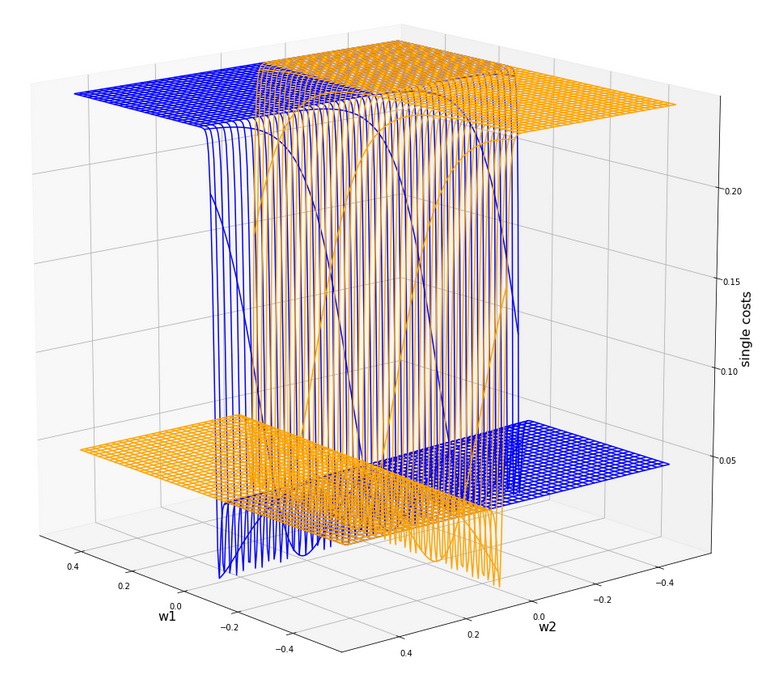Actually the cost functions for the different samples do show some systematic, though small differences. Try it out yourself ... Here is the plot for samples 1,5,9 (counted from 0!).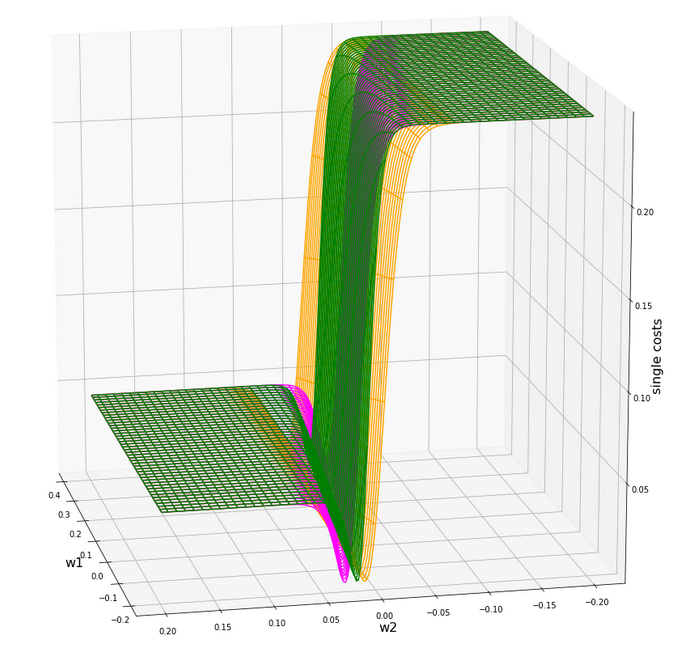You see different orientation angles in the (w1, w2)-plane?

# Total cost landscape without normalization

Now let us look at the total costs; to arrive at a comparable level with the other plots I divided the sum by 14: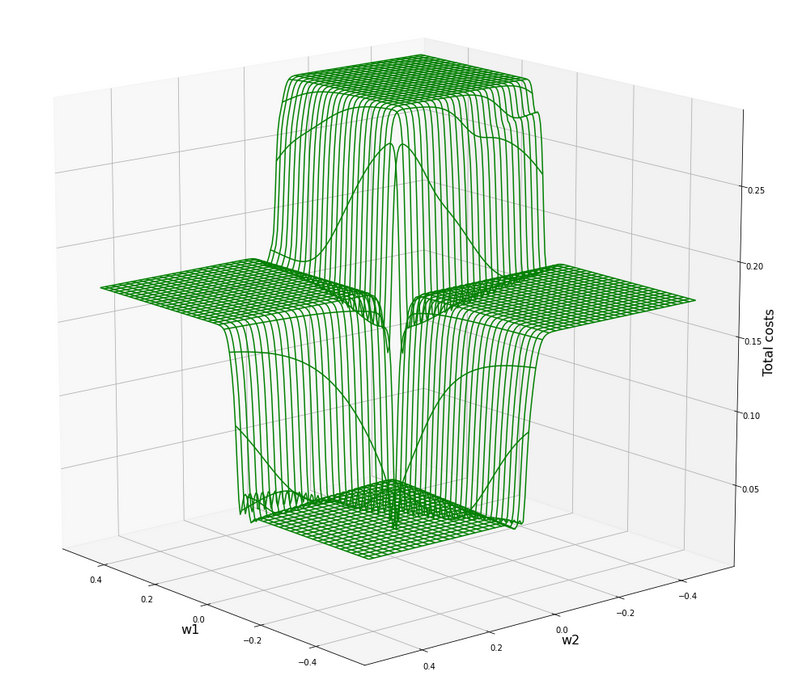All of the above cost plots look like big trouble for both the "stochastic gradient descent" and the "batch gradient descent" methods for "Machine Learning" [ML]:

We clearly see the effect of the sigmoid saturation. We get almost flat areas beyond certain relatively small w1- and w2-values (|w1| > 0.02, |w2| > 0.02). The gradients in this areas will be very, very close to zero. So, if we have a starting point as e.g. (w1=0.3, w2=0.2) our gradient descent would get stuck. Due to the big input values of at least one feature.

In the center of the {w1, w2}-plane, however, we detect a steep slope to a global minimum.

But how to get there? Let us say, we start with w1=0.04, w2=0.04. The learning-rate "η" is used to correct the weight values by

In the beginning you would need a big "η" to get closer to the center due to small gradient values. However, if you choose it too big you may pass the tiny area of the minimum and just hop to an area of a different cost level with again a gradient close to zero. But you cannot decrease the learning rate fast as a remedy, either, to avoid getting stuck again.

# A view at the center of the loss function hyperplane

Let us take a closer look at the center of our disastrous total cost function. We can get there by reducing our mesh to a region defined by "-0.05 < w1, w2 < 0.05". We get :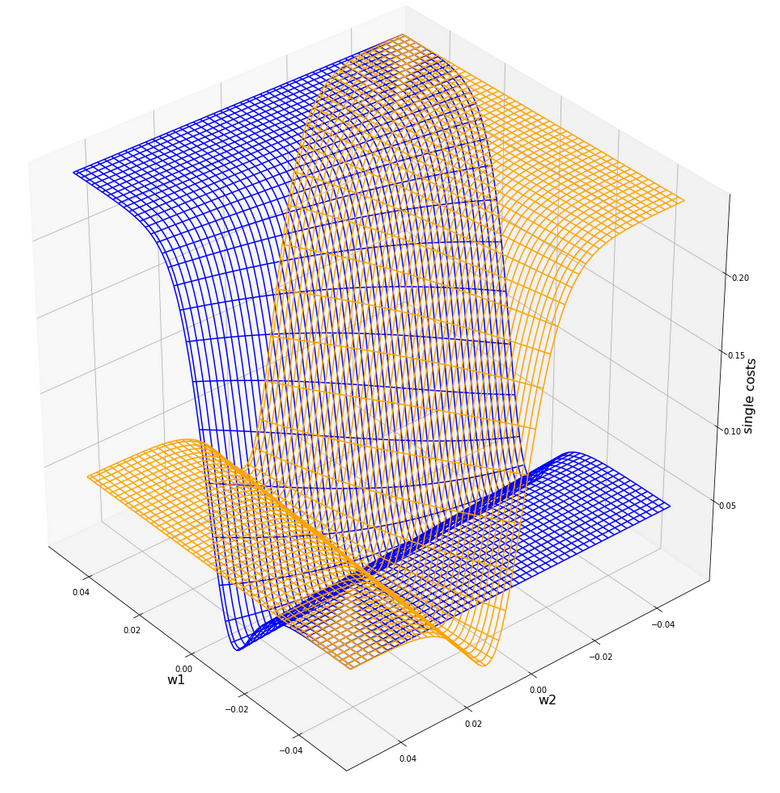This looks actually much better - on such a surface we could probably work with gradient descent. There is a clear minimum visible - and on this scale of the (w1, w2)-values we also recognize reasonably paths into this minimum. An analysis of the meshdata to get values for the minimum is possible by the following statements:

```print("min =", C.min())
pt_min = np.argwhere(C==C.min())
w1=W1[pt_min][pt_min]
w2=W2[pt_min][pt_min]
print("w1 = ", w1)
print("w2 = ", w2)
```

The result is:

min = 0.0006446277000906343
w1 = -0.004999999999999963
w2 = 0.005000000000000046

But to approach this minimum by a smooth gradient descent we would have had to know in advance at what tiny values of (w1, w2) to start with gradient descent - and choose our η suitably small in addition. This is easy in our most simplistic one neuron case, but you almost never can fulfill the first condition when dealing with real artificial neural networks for complex scenarios.

And a naive gradient descent with a standard choice of a (w1, w2)-starting point would have lead us to nowhere in our one-neuron case - as we shall see in a minute ..

Let us keep one question in mind for a while: Is there a chance that we could get the hyperplane surface to look similar to the one at the center - but for much bigger weight values?

# Some Python code for gradient descent for our one neuron scenario

Here are some useful functions, which we shall use later on to perform a gradient descent:

```
# ****************************************
# Functions for stochastic GRADIENT DESCENT
# *****************************************
import random
import pandas as pd

# derivative of expit
def d_expit(z):
exz = expit(z)
dex = exz * (1.0 - exz)
return dex

# single costs for stochastic descent
# ************************************
def dcost_sgl(w1, w2, idx, li_K1, li_K2, li_a_tgt):
z_in  = w1 * li_K1[idx] + w2 * li_K2[idx]
a_tgt = li_a_tgt[idx]
c = 0.5 * ( a_tgt - expit(z_in))**2
return c

# *********
def grad_sgl(w1, w2, idx, li_K1, li_K2, li_a_tgt):
z_in  = w1 * li_K1[idx] + w2 * li_K2[idx]
a_tgt = li_a_tgt[idx]
gradw1 = 0.5 * 2.0 * (a_tgt - expit(z_in)) * (-d_expit(z_in)) * li_K1[idx]
gradw2 = 0.5 * 2.0 * (a_tgt - expit(z_in)) * (-d_expit(z_in)) * li_K2[idx]

def grad_tot(num_samples, w1, w2, li_K1, li_K2, li_a_tgt):
rg_idx = range(num_samples)
for idx in rg_idx:
z_in  = w1 * li_K1[idx] + w2 * li_K2[idx]
a_tgt = li_a_tgt[idx]
gradw1_idx = 0.5 * 2.0 * (a_tgt - expit(z_in)) * (-d_expit(z_in)) * li_K1[idx]
gradw2_idx = 0.5 * 2.0 * (a_tgt - expit(z_in)) * (-d_expit(z_in)) * li_K2[idx]

# total costs at given point
# ************************************
def dcost_tot(num_samples, w1, w2,li_K1, li_K2, li_a_tgt):
c_tot  = 0
rg_idx = range(num_samples)
for idx in rg_idx:
#z_in  = w1 * li_K1[idx] + w2 * li_K2[idx]
a_tgt = li_a_tgt[idx]
c_idx = dcost_sgl(w1, w2, idx, li_K1, li_K2, li_a_tgt)
c_tot += c_idx
ctot = 1.0/num_samples * c_tot
return c_tot

# Prediction function
# ********************
def predict_batch(num_samples, w1, w2,ay_k_1, ay_k_2, li_a_tgt):
shape_res = (num_samples, 5)
ResData = np.zeros(shape_res)
rg_idx = range(num_samples)
err = 0.0
for idx in rg_idx:
z_in  = w1 * ay_k_1[idx] + w2 * ay_k_2[idx]
a_out = expit(z_in)
a_tgt = li_a_tgt[idx]
err_idx = np.absolute(a_out - a_tgt) / a_tgt
err += err_idx
ResData[idx] = ay_k_1[idx]
ResData[idx] = ay_k_2[idx]
ResData[idx] = a_tgt
ResData[idx] = a_out
ResData[idx] = err_idx
err /= float(num_samples)
return err, ResData

def predict_sgl(k1, k2, w1, w2):
z_in  = w1 * k1 + w2 * k2
a_out = expit(z_in)
return a_out

def create_df(ResData):
''' ResData: Array with result values K1, K2, Tgt, A, rel.err
'''
cols=["K1", "K2", "Tgt", "Res", "Err"]
df = pd.DataFrame(ResData, columns=cols)
return df

```

With these functions a quick and dirty "gradient descent" can be achieved by the following code:

```
# **********************************
# Quick and dirty Gradient Descent
# **********************************
b_scale_2 = False
if b_scale_2:
ay_k_1 = ay_K1
ay_k_2 = ay_K2
else:
ay_k_1 = li_K1
ay_k_2 = li_K2

li_w1_st = []
li_w2_st = []
li_c_sgl_st = []
li_c_tot_st = []

li_w1_ba = []
li_w2_ba = []
li_c_sgl_ba = []
li_c_tot_ba = []

idxc = 2

# Starting point
#***************
w1_start = -0.04
w2_start = -0.0455
#w1_start = 0.5
#w2_start = -0.5

# learn rate
# **********
eta = 0.0001
decrease_rate = 0.000000001
num_steps = 2500

# norm = 1
#eta = 0.75
#decrease_rate = 0.000000001
#num_steps = 100

# *********************
rg_j = range(num_steps)
rg_i = range(num_samples)
w1d_st = w1_start
w2d_st = w2_start
w1d_ba = w1_start
w2d_ba = w2_start

for j in rg_j:
eta = eta / (1.0 + float(j) * decrease_rate)
# loop over samples and individ. corrs
ns = num_samples
rg = range(ns)
rg_idx = random.sample(rg, num_samples)
#print("\n")
for idx in rg_idx:
#print("idx = ", idx)
li_w1_st.append(w1d_st)
li_w2_st.append(w2d_st)

# costs for special sample
cd_sgl_st = dcost_sgl(w1d_st, w2d_st, idx, ay_k_1, ay_k_2, li_a_tgt)
li_c_sgl_st.append(cd_sgl_st)

# total costs for special sample
cd_tot_st = dcost_tot(num_samples, w1d_st, w2d_st, ay_k_1, ay_k_2, li_a_tgt)
li_c_tot_st.append(cd_tot_st)
#print("j:", j, " li_c_tot[j] = ", li_c_tot[j] )

# work with total costs and total gradient
li_w1_ba.append(w1d_ba)
li_w2_ba.append(w2d_ba)
co_ba = dcost_tot(num_samples, w1d_ba, w2d_ba, ay_k_1, ay_k_2, li_a_tgt)
li_c_tot_ba.append(co_ba)

# Printed Output
# ***************
num_end = len(li_w1_st)
err_sgl, ResData_sgl = predict_batch(num_samples, li_w1_st[num_end-1], li_w2_st[num_end-1], ay_k_1, ay_k_2, li_a_tgt)
err_ba,  ResData_ba = predict_batch(num_samples, li_w1_ba[num_steps-1], li_w2_ba[num_steps-1], ay_k_1, ay_k_2, li_a_tgt)
df_sgl = create_df(ResData_sgl)
df_ba  = create_df(ResData_ba)
print("\n", df_sgl)
print("\n", df_ba)
print("\nTotal error stoch descent: ", err_sgl )
print("Total error batch descent: ", err_ba )

# Styled Pandas Output
# *******************
df_ba

```

Those readers who followed my series on a Multilayer Perceptron should have no difficulties to understand the code: I used two methods in parallel - one for a "stochastic descent" and one for a "batch descent":

• During "stochastic descent" we correct the weights by a stepwise application of the cost-gradients of single samples. (We shuffle the order of the samples statistically during epochs to avoid cyclic effects.) This is done for all samples during an epoch.
• During batch gradient we apply the gradient of the total costs of all samples once during each epoch.

And here is also some code to perform some plotting after training runs:

```
# Plots for Single neuron Gradient Descent
# ****************************************
#sizing
fig_size = plt.rcParams["figure.figsize"]
fig_size = 14
fig_size = 5

fig1 = plt.figure(1)
fig2 = plt.figure(2)
fig3 = plt.figure(3)
fig4 = plt.figure(4)

ax1_1.plot(range(len(li_c_sgl_st)), li_c_sgl_st)
#ax1_1.set_xlim (0, num_tot+5)
#ax1_1.set_ylim (0, 0.4)
ax1_1.set_xlabel("epochs * batches (" + str(num_steps) + " * " + str(num_samples) + " )")
ax1_1.set_ylabel("sgl costs")

ax1_2.plot(range(len(li_c_tot_st)), li_c_tot_st)
#ax1_2.set_xlim (0, num_tot+5)
#ax1_2.set_ylim (0, y_max_err)
ax1_2.set_xlabel("epochs * batches (" + str(num_steps) + " * " + str(num_samples) + " )")
ax1_2.set_ylabel("total costs ")

ax2_1.plot(range(len(li_w1_st)), li_w1_st)
#ax1_1.set_xlim (0, num_tot+5)
#ax1_1.set_ylim (0, y_max_costs)
ax2_1.set_xlabel("epochs * batches (" + str(num_steps) + " * " + str(num_samples) + " )")
ax2_1.set_ylabel("w1")

ax2_2.plot(range(len(li_w2_st)), li_w2_st)
#ax1_2.set_xlim (0, num_to_t+5)
#ax1_2.set_ylim (0, y_max_err)
ax2_2.set_xlabel("epochs * batches (" + str(num_steps) + " * " + str(num_samples) + " )")
ax2_2.set_ylabel("w2")

ax3_1.plot(range(len(li_c_tot_ba)), li_c_tot_ba)
#ax3_1.set_xlim (0, num_tot+5)
#ax3_1.set_ylim (0, 0.4)
ax3_1.set_xlabel("epochs (" + str(num_steps) + " )")
ax3_1.set_ylabel("batch costs")

ax4_1.plot(range(len(li_w1_ba)), li_w1_ba)
#ax4_1.set_xlim (0, num_tot+5)
#ax4_1.set_ylim (0, y_max_costs)
ax4_1.set_xlabel("epochs (" + str(num_steps) + " )")
ax4_1.set_ylabel("w1")

ax4_2.plot(range(len(li_w2_ba)), li_w2_ba)
#ax4_2.set_xlim (0, num_to_t+5)
#ax4_2.set_ylim (0, y_max_err)
ax4_2.set_xlabel("epochs (" + str(num_steps) + " )")
ax4_2.set_ylabel("w2")

```

You can put these codes into suitable cells of a Jupyter environment and start doing experiments on your PC.

# Frustration WITHOUT data normalization ...

Let us get the worst behind us:
Let us use un-normalized input data, set a standard starting point for the weights and try a gradient descent with 2500 epochs.
Well, what are standard initial weight values? We can follow LeCun's advice on bigger networks: a uniform distribution between - sqrt(1/2) and +srt(1/2) = 0.7 should be helpful. Well, we take such values. The parameters of our trial run are:

w1_start = -0.1, w2_start = 0.1, eta = 0.01, decrease_rate = 0.000000001, num_steps = 12500

You, unfortunately, get nowhere:

```        K1     K2  Tgt           Res       Err
0   200.0   14.0  0.3  3.124346e-15  1.000000
1     1.0  107.0  0.7  9.999996e-01  0.428571
2   160.0   10.0  0.3  2.104822e-12  1.000000
3    11.0  193.0  0.7  1.000000e+00  0.428571
4   220.0   32.0  0.3  1.117954e-15  1.000000
5    11.0  178.0  0.7  1.000000e+00  0.428571
6   120.0    2.0  0.3  8.122661e-10  1.000000
7    14.0  210.0  0.7  1.000000e+00  0.428571
8   195.0   12.0  0.3  5.722374e-15  1.000000
9    15.0  134.0  0.7  9.999999e-01  0.428571
10  130.0    7.0  0.3  2.783284e-10  1.000000
11    5.0  167.0  0.7  1.000000e+00  0.428571
12  185.0   10.0  0.3  2.536279e-14  1.000000
13   16.0  229.0  0.7  1.000000e+00  0.428571

K1     K2  Tgt           Res       Err
0   200.0   14.0  0.3  7.567897e-24  1.000000
1     1.0  107.0  0.7  1.000000e+00  0.428571
2   160.0   10.0  0.3  1.485593e-19  1.000000
3    11.0  193.0  0.7  1.000000e+00  0.428571
4   220.0   32.0  0.3  1.411189e-21  1.000000
5    11.0  178.0  0.7  1.000000e+00  0.428571
6   120.0    2.0  0.3  2.293804e-16  1.000000
7    14.0  210.0  0.7  1.000000e+00  0.428571
8   195.0   12.0  0.3  1.003437e-23  1.000000
9    15.0  134.0  0.7  1.000000e+00  0.428571
10  130.0    7.0  0.3  2.463730e-16  1.000000
11    5.0  167.0  0.7  1.000000e+00  0.428571
12  185.0   10.0  0.3  6.290055e-23  1.000000
13   16.0  229.0  0.7  1.000000e+00  0.428571

Total error stoch descent:  0.7142856616691734
Total error batch descent:  0.7142857142857143
```

A parameter setting like

w1_start = -0.1, w2_start = 0.1, eta = 0.0001, decrease_rate = 0.000000001, num_steps = 25000

does not bring us any further:

```        K1     K2  Tgt           Res       Err
0   200.0   14.0  0.3  9.837323e-09  1.000000
1     1.0  107.0  0.7  9.999663e-01  0.428523
2   160.0   10.0  0.3  3.496673e-07  0.999999
3    11.0  193.0  0.7  1.000000e+00  0.428571
4   220.0   32.0  0.3  7.812207e-09  1.000000
5    11.0  178.0  0.7  9.999999e-01  0.428571
6   120.0    2.0  0.3  8.425742e-06  0.999972
7    14.0  210.0  0.7  1.000000e+00  0.428571
8   195.0   12.0  0.3  1.328667e-08  1.000000
9    15.0  134.0  0.7  9.999902e-01  0.428557
10  130.0    7.0  0.3  5.090220e-06  0.999983
11    5.0  167.0  0.7  9.999999e-01  0.428571
12  185.0   10.0  0.3  2.943780e-08  1.000000
13   16.0  229.0  0.7  1.000000e+00  0.428571

K1     K2  Tgt           Res       Err
0   200.0   14.0  0.3  9.837323e-09  1.000000
1     1.0  107.0  0.7  9.999663e-01  0.428523
2   160.0   10.0  0.3  3.496672e-07  0.999999
3    11.0  193.0  0.7  1.000000e+00  0.428571
4   220.0   32.0  0.3  7.812208e-09  1.000000
5    11.0  178.0  0.7  9.999999e-01  0.428571
6   120.0    2.0  0.3  8.425741e-06  0.999972
7    14.0  210.0  0.7  1.000000e+00  0.428571
8   195.0   12.0  0.3  1.328667e-08  1.000000
9    15.0  134.0  0.7  9.999902e-01  0.428557
10  130.0    7.0  0.3  5.090220e-06  0.999983
11    5.0  167.0  0.7  9.999999e-01  0.428571
12  185.0   10.0  0.3  2.943780e-08  1.000000
13   16.0  229.0  0.7  1.000000e+00  0.428571

Total error stoch descent:  0.7142779420120247
Total error batch descent:  0.7142779420164836
```

However:
For the following parameters we do get something:

w1_start = -0.1, w2_start = 0.1, eta = 0.001, decrease_rate = 0.000000001, num_steps = 25000

```        K1     K2  Tgt       Res       Err
0   200.0   14.0  0.3  0.298207  0.005976
1     1.0  107.0  0.7  0.603422  0.137969
2   160.0   10.0  0.3  0.334158  0.113860
3    11.0  193.0  0.7  0.671549  0.040644
4   220.0   32.0  0.3  0.294089  0.019705
5    11.0  178.0  0.7  0.658298  0.059574
6   120.0    2.0  0.3  0.368446  0.228154
7    14.0  210.0  0.7  0.683292  0.023869
8   195.0   12.0  0.3  0.301325  0.004417
9    15.0  134.0  0.7  0.613729  0.123244
10  130.0    7.0  0.3  0.362477  0.208256
11    5.0  167.0  0.7  0.654627  0.064819
12  185.0   10.0  0.3  0.309307  0.031025
13   16.0  229.0  0.7  0.697447  0.003647

K1     K2  Tgt       Res       Err
0   200.0   14.0  0.3  0.000012  0.999961
1     1.0  107.0  0.7  0.997210  0.424586
2   160.0   10.0  0.3  0.000106  0.999646
3    11.0  193.0  0.7  0.999957  0.428510
4   220.0   32.0  0.3  0.000009  0.999968
5    11.0  178.0  0.7  0.999900  0.428429
6   120.0    2.0  0.3  0.000771  0.997429
7    14.0  210.0  0.7  0.999980  0.428543
8   195.0   12.0  0.3  0.000014  0.999953
9    15.0  134.0  0.7  0.998541  0.426487
10  130.0    7.0  0.3  0.000555  0.998150
11    5.0  167.0  0.7  0.999872  0.428389
12  185.0   10.0  0.3  0.000023  0.999922
13   16.0  229.0  0.7  0.999992  0.428560

Total error single:  0.07608269490258893
Total error batch:  0.7134665897677123
```

By pure chance we found a combination of starting point and learning-rate for which we - by hopping around on the flat cost areas - we accidentally arrived at the slope area of one sample and started a gradient descent. This did however not (yet) happen for the total costs.
We get a minimum around (w1=-0.005,w2=0.005) but with a big spread of 0.0025 for each of the weight values.

# Intermediate Conclusion

We looked at a simple perceptron scenario with one computing neuron. Our solitary neuron should learn to distinguish between input data of two distinct and separate data clusters in a 2-dimensional feature space. The feature data change between big and small values for different samples. The neuron used the sigmoid-function as activation and output function. The cost function for all samples shows a minimum at a tiny area in the weight space. We found this minimum with the help of a fine grained and mesh-based analysis of the cost values. However, such an analysis is not applicable to general ML-scenarios.

The problem we face is that due to the saturation properties of the sigmoid function the minimum cannot be detected automatically via gradient descent without knowing already precise details about the solution. Gradient descent does not work - we either get stuck on areas of nearly constant costs or we hop around between different plateaus of the cost function - missing a tiny location in the (w1, w2)-parameter space for a real descent into the existing minimum.

We need to find a way out of this dilemma. In the next article

A single neuron perceptron with sigmoid activation function – II – normalization to overcome saturation

I shall show that normalization opens such a way.